# The seaborn.objects interface#

The `seaborn.objects` namespace was introduced in version 0.12 as a completely new interface for making seaborn plots. It offers a more consistent and flexible API, comprising a collection of composable classes for transforming and plotting data. In contrast to the existing `seaborn` functions, the new interface aims to support end-to-end plot specification and customization without dropping down to matplotlib (although it will remain possible to do so if necessary).

Note

The objects interface is currently experimental and incomplete. It is stable enough for serious use, but there certainly are some rough edges and missing features.

## Specifying a plot and mapping data#

The objects interface should be imported with the following convention:

```import seaborn.objects as so
```

The `seaborn.objects` namespace will provide access to all of the relevant classes. The most important is `Plot`. You specify plots by instantiating a `Plot` object and calling its methods. Let’s see a simple example:

```(
so.Plot(penguins, x="bill_length_mm", y="bill_depth_mm")
)
```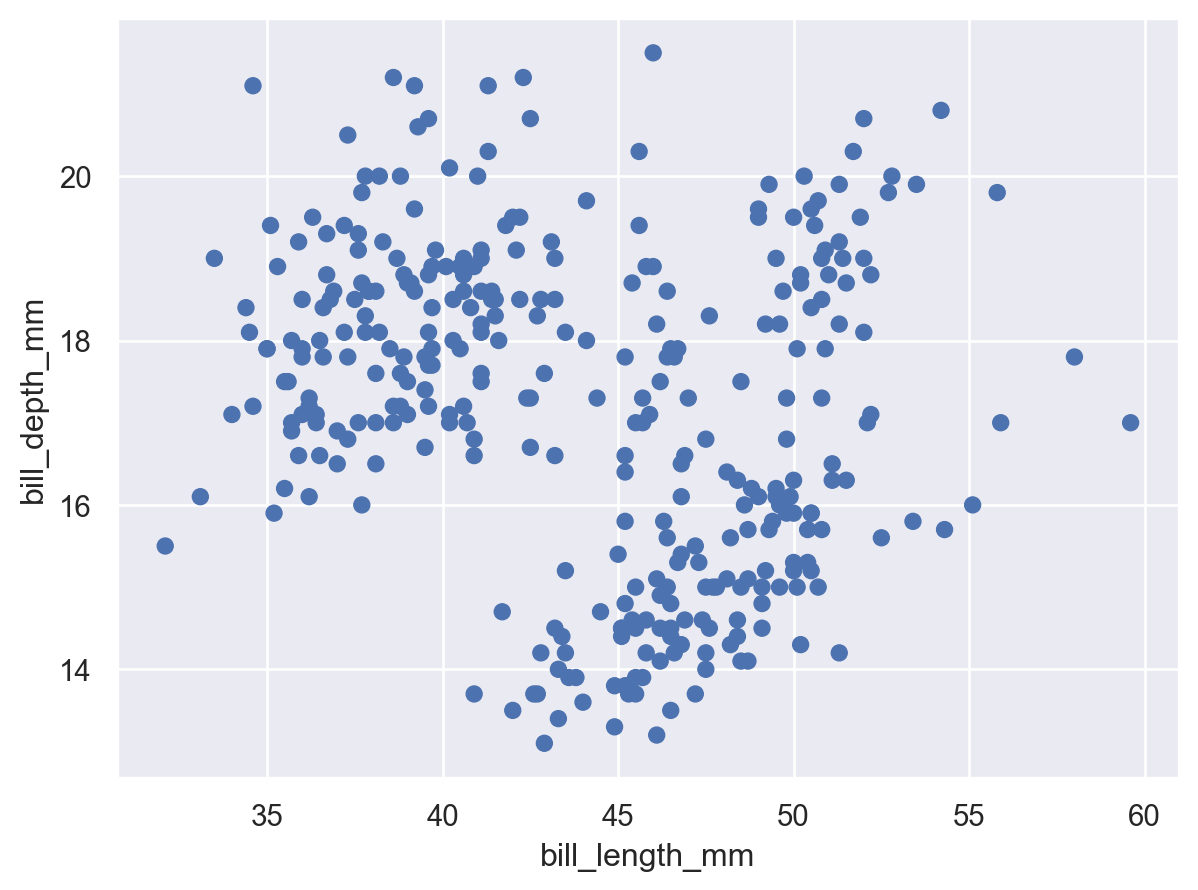This code, which produces a scatter plot, should look reasonably familiar. Just as when using `seaborn.scatterplot()`, we passed a tidy dataframe (`penguins`) and assigned two of its columns to the `x` and `y` coordinates of the plot. But instead of starting with the type of chart and then adding some data assignments, here we started with the data assignments and then added a graphical element.

### Setting properties#

The `Dot` class is an example of a `Mark`: an object that graphically represents data values. Each mark will have a number of properties that can be set to change its appearance:

```(
so.Plot(penguins, x="bill_length_mm", y="bill_depth_mm")
)
```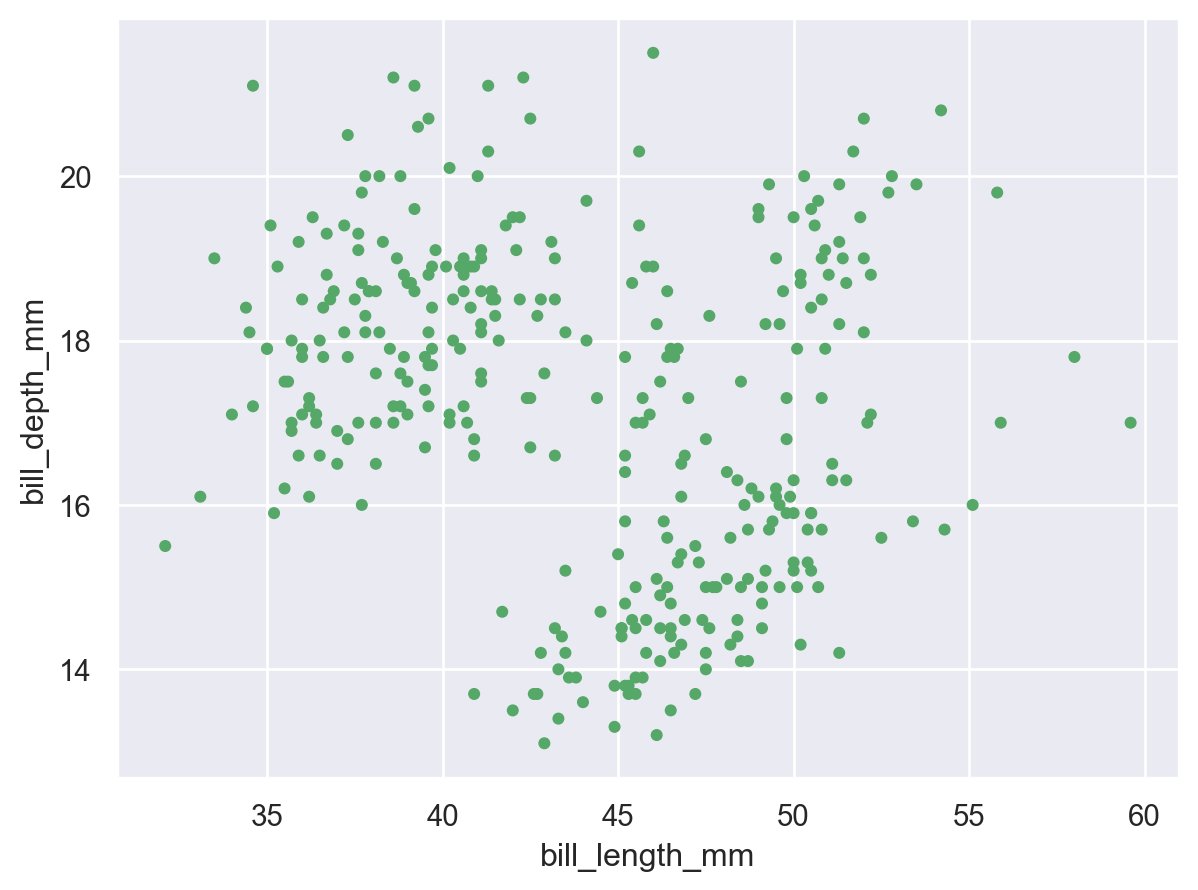### Mapping properties#

As with seaborn’s functions, it is also possible to map data values to various graphical properties:

```(
so.Plot(
penguins, x="bill_length_mm", y="bill_depth_mm",
color="species", pointsize="body_mass_g",
)
)
```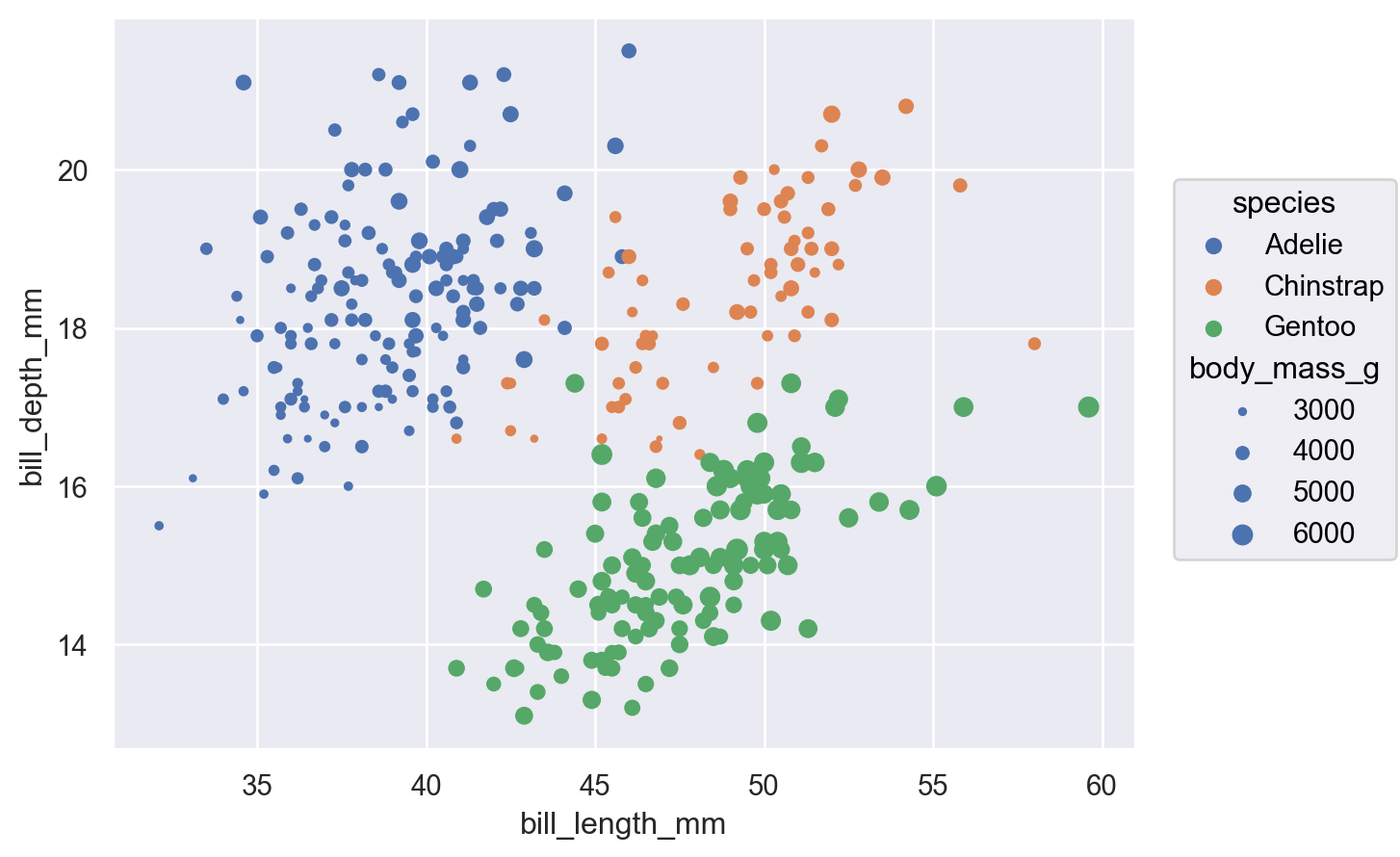While this basic functionality is not novel, an important difference from the function API is that properties are mapped using the same parameter names that would set them directly (instead of having `hue` vs. `color`, etc.). What matters is where the property is defined: passing a value when you initialize `Dot` will set it directly, whereas assigning a variable when you set up the `Plot` will map the corresponding data.

Beyond this difference, the objects interface also allows a much wider range of mark properties to be mapped:

```(
so.Plot(
penguins, x="bill_length_mm", y="bill_depth_mm",
edgecolor="sex", edgewidth="body_mass_g",
)
)
```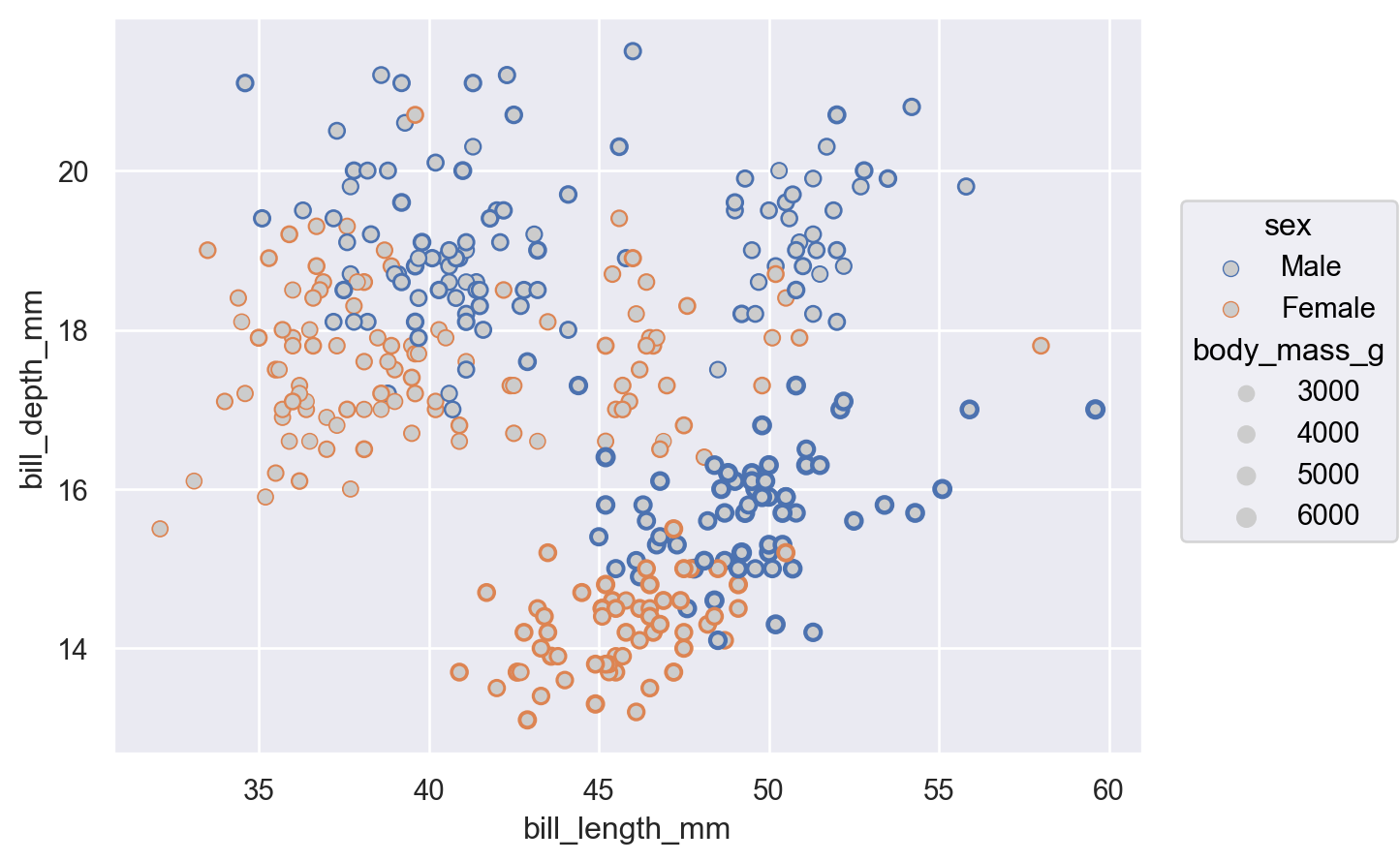### Defining groups#

The `Dot` mark represents each data point independently, so the assignment of a variable to a property only has the effect of changing each dot’s appearance. For marks that group or connect observations, such as `Line`, it also determines the number of distinct graphical elements:

```(
so.Plot(healthexp, x="Year", y="Life_Expectancy", color="Country")
)
```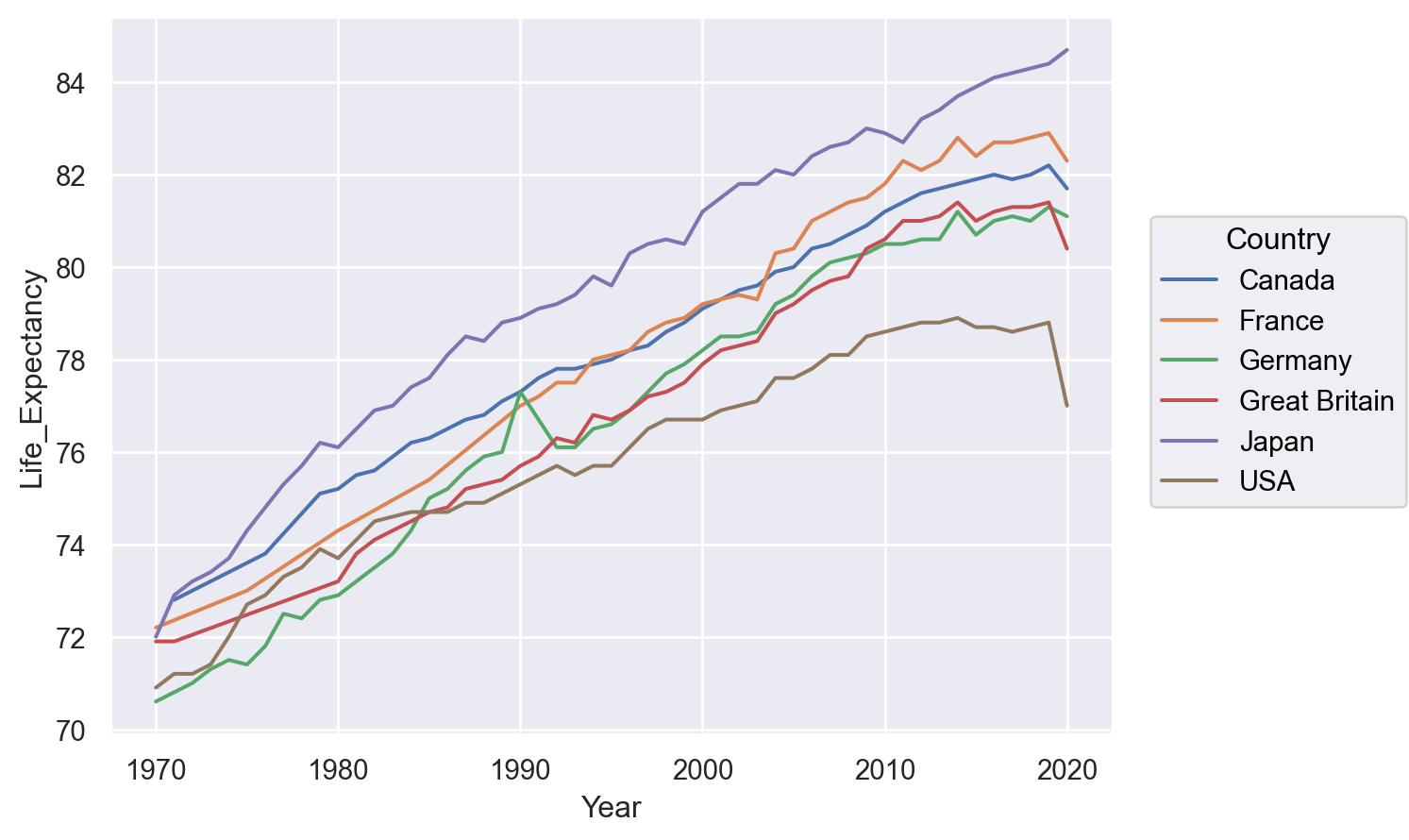It is also possible to define a grouping without changing any visual properties, by using `group`:

```(
so.Plot(healthexp, x="Year", y="Life_Expectancy", group="Country")
)
```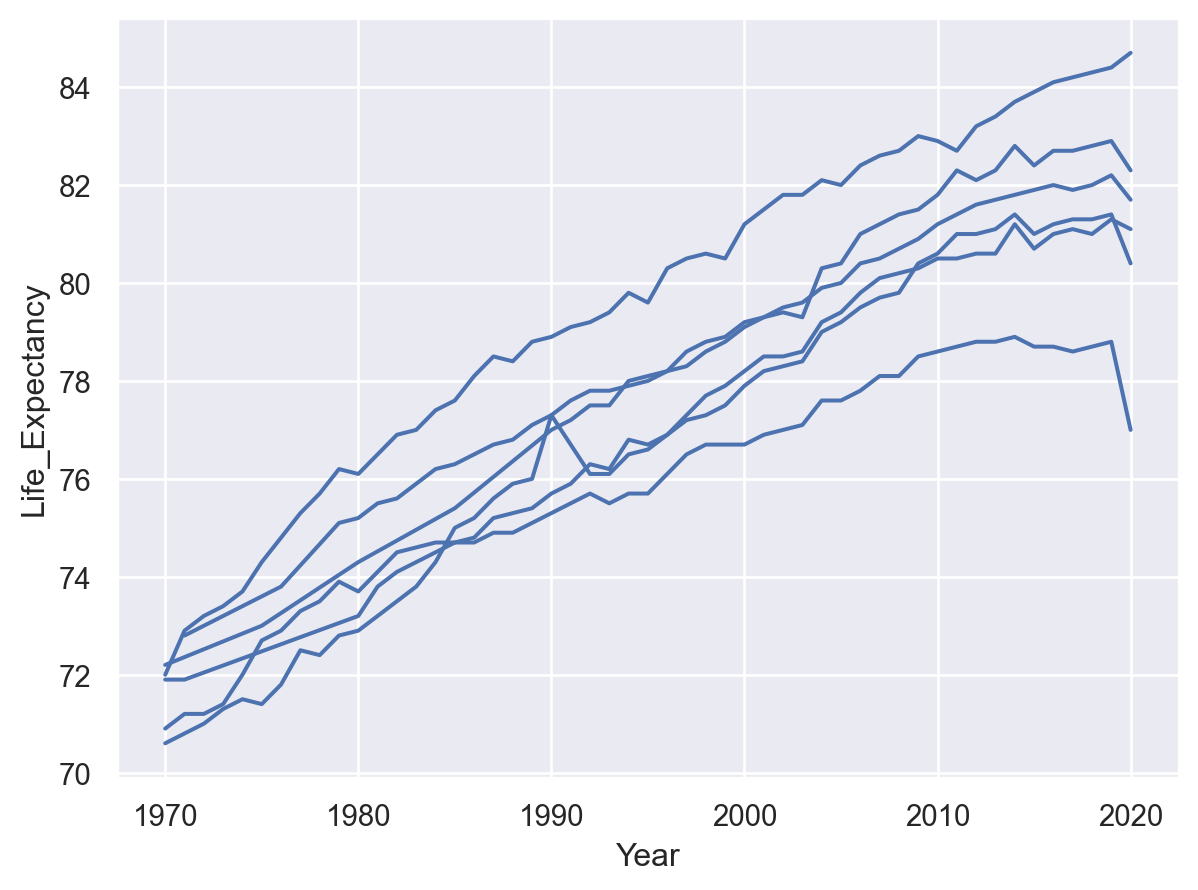## Transforming data before plotting#

### Statistical transformation#

As with many seaborn functions, the objects interface supports statistical transformations. These are performed by `Stat` objects, such as `Agg`:

```(
so.Plot(penguins, x="species", y="body_mass_g")
)
```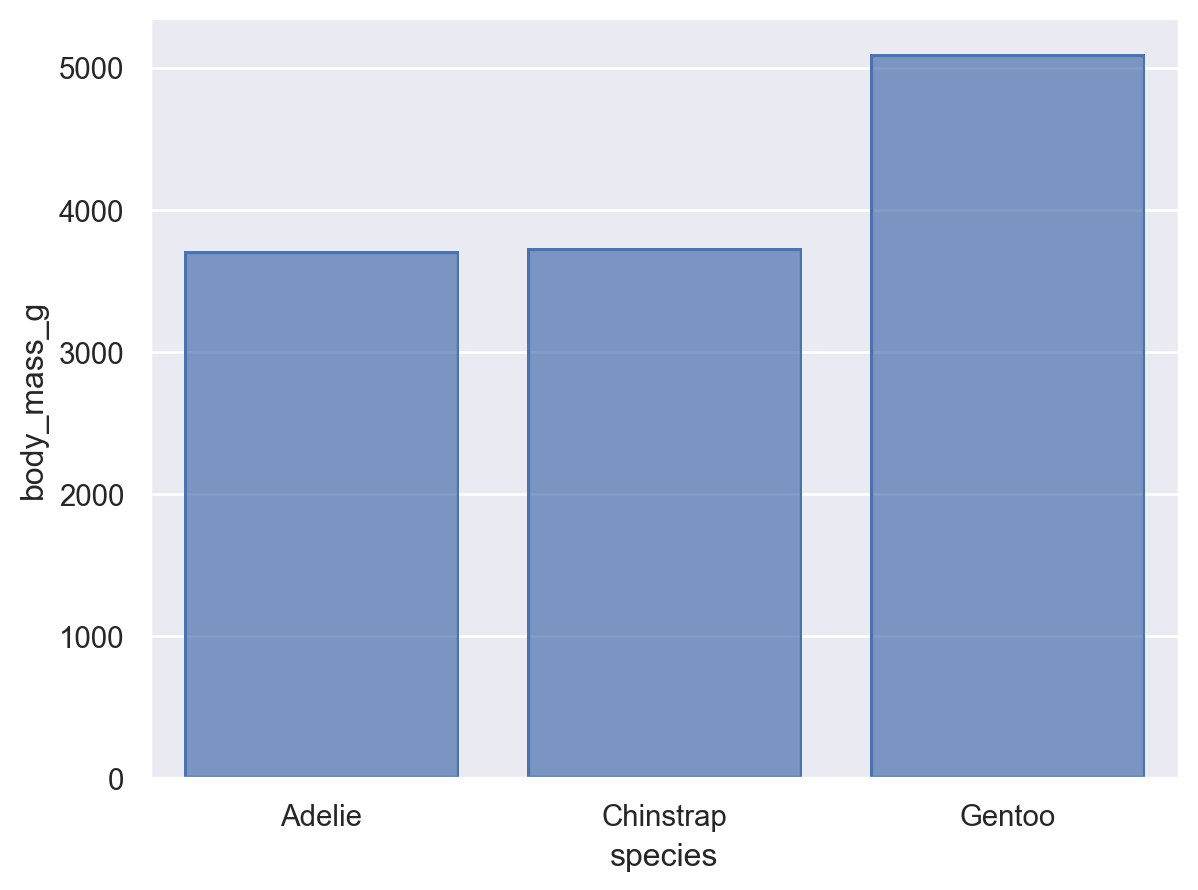In the function interface, statistical transformations are possible with some visual representations (e.g. `seaborn.barplot()`) but not others (e.g. `seaborn.scatterplot()`). The objects interface more cleanly separates representation and transformation, allowing you to compose `Mark` and `Stat` objects:

```(
so.Plot(penguins, x="species", y="body_mass_g")
)
```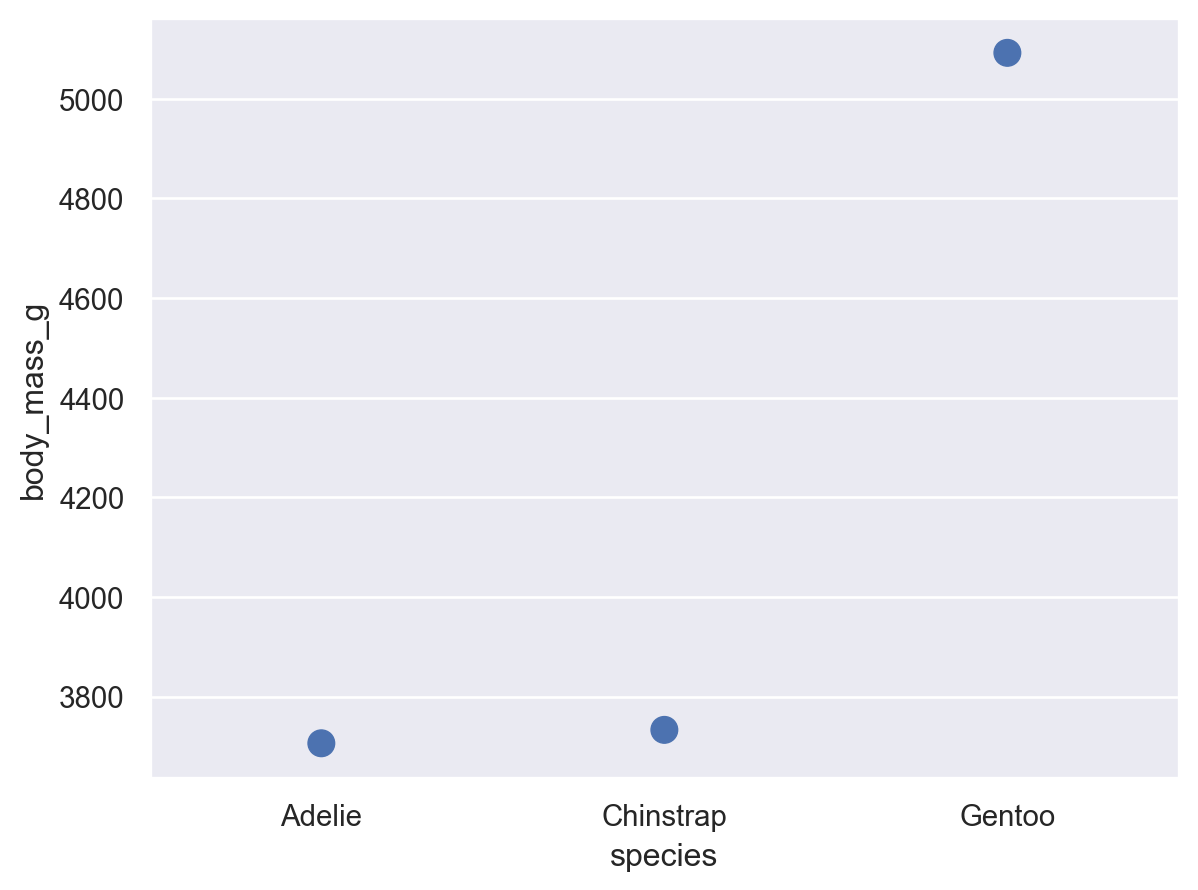When forming groups by mapping properties, the `Stat` transformation is applied to each group separately:

```(
so.Plot(penguins, x="species", y="body_mass_g", color="sex")
)
```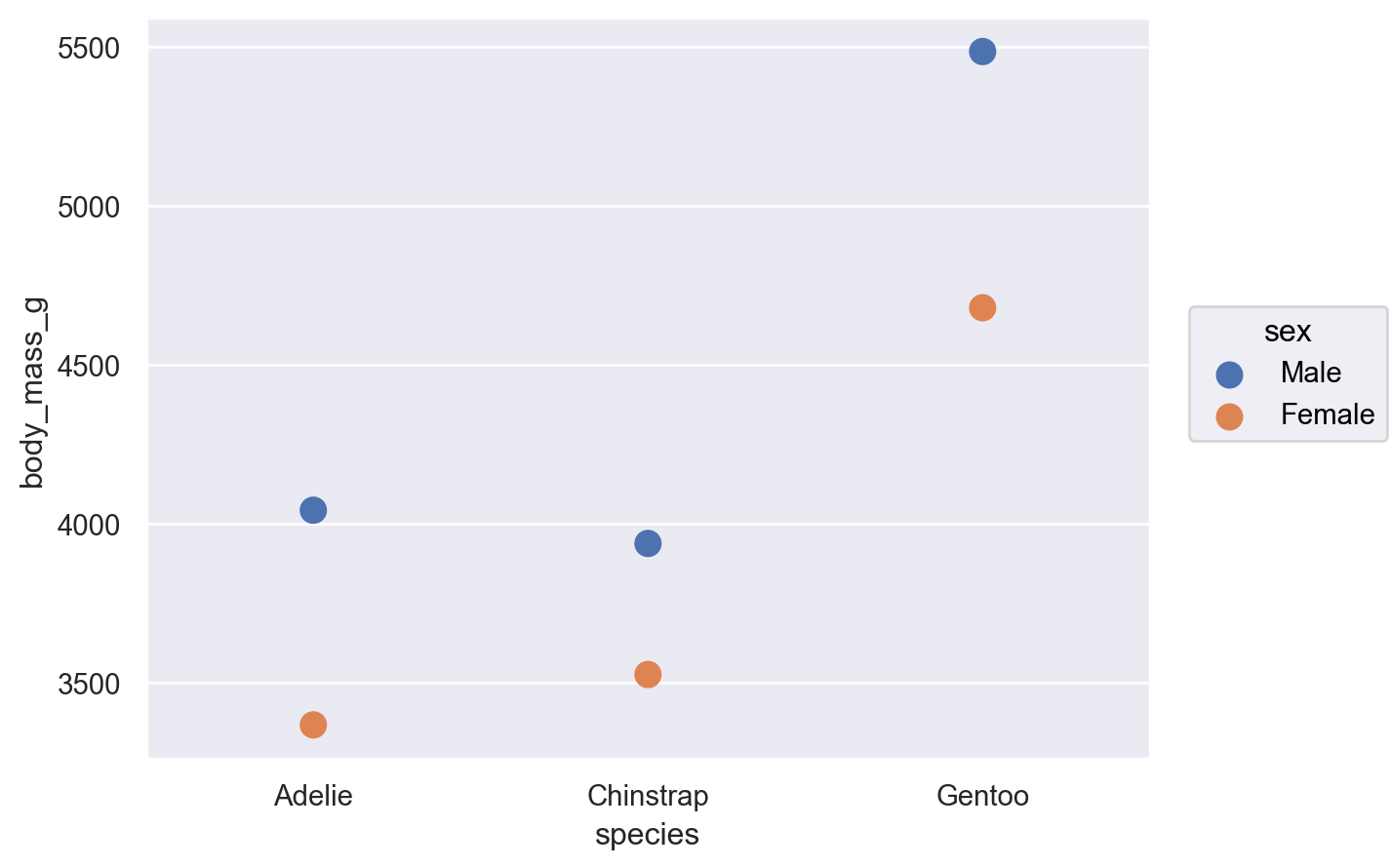### Resolving overplotting#

Some seaborn functions also have mechanisms that automatically resolve overplotting, as when `seaborn.barplot()` “dodges” bars once `hue` is assigned. The objects interface has less complex default behavior. Bars representing multiple groups will overlap by default:

```(
so.Plot(penguins, x="species", y="body_mass_g", color="sex")
)
```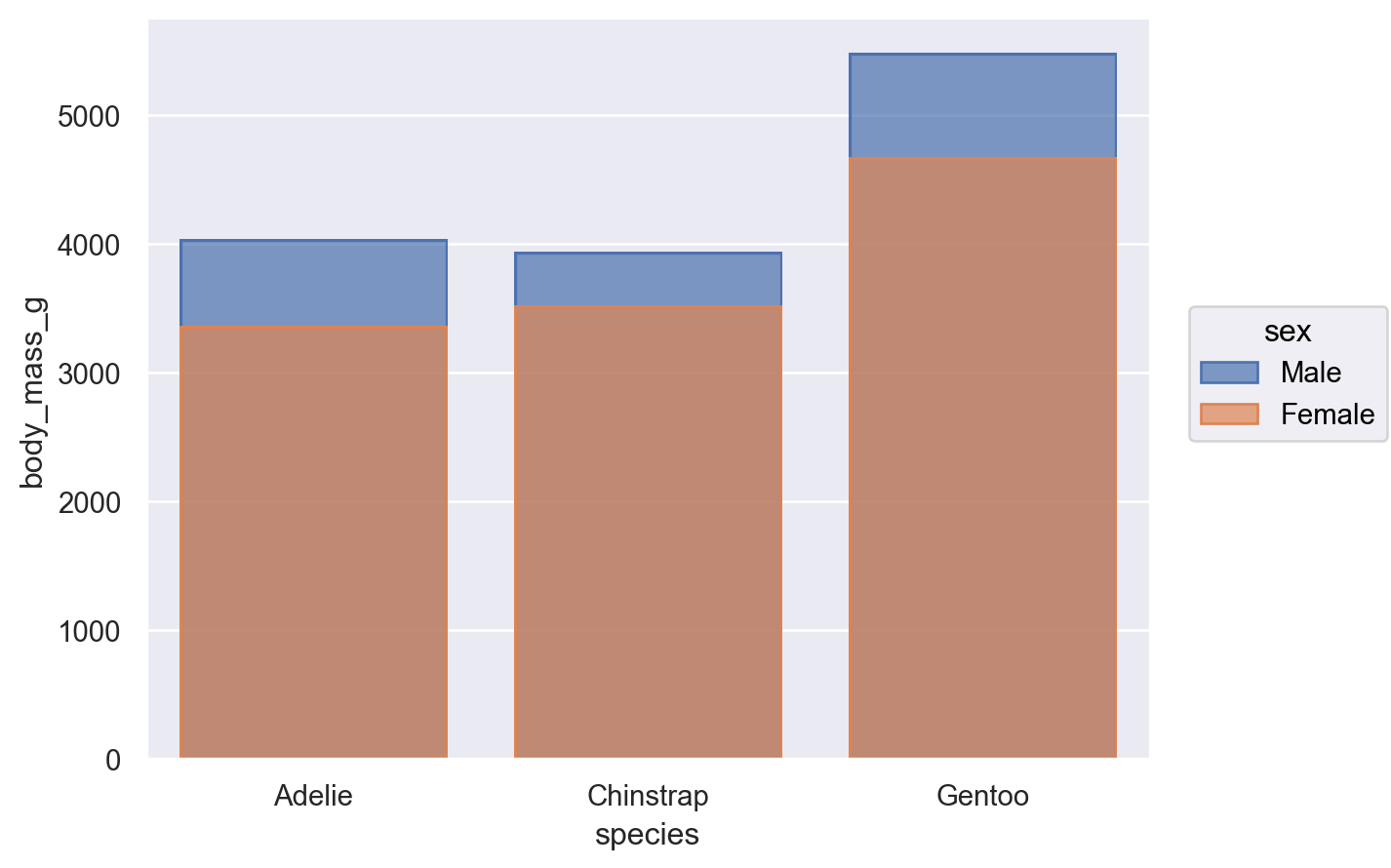Nevertheless, it is possible to compose the `Bar` mark with the `Agg` stat and a second transformation, implemented by `Dodge`:

```(
so.Plot(penguins, x="species", y="body_mass_g", color="sex")
)
```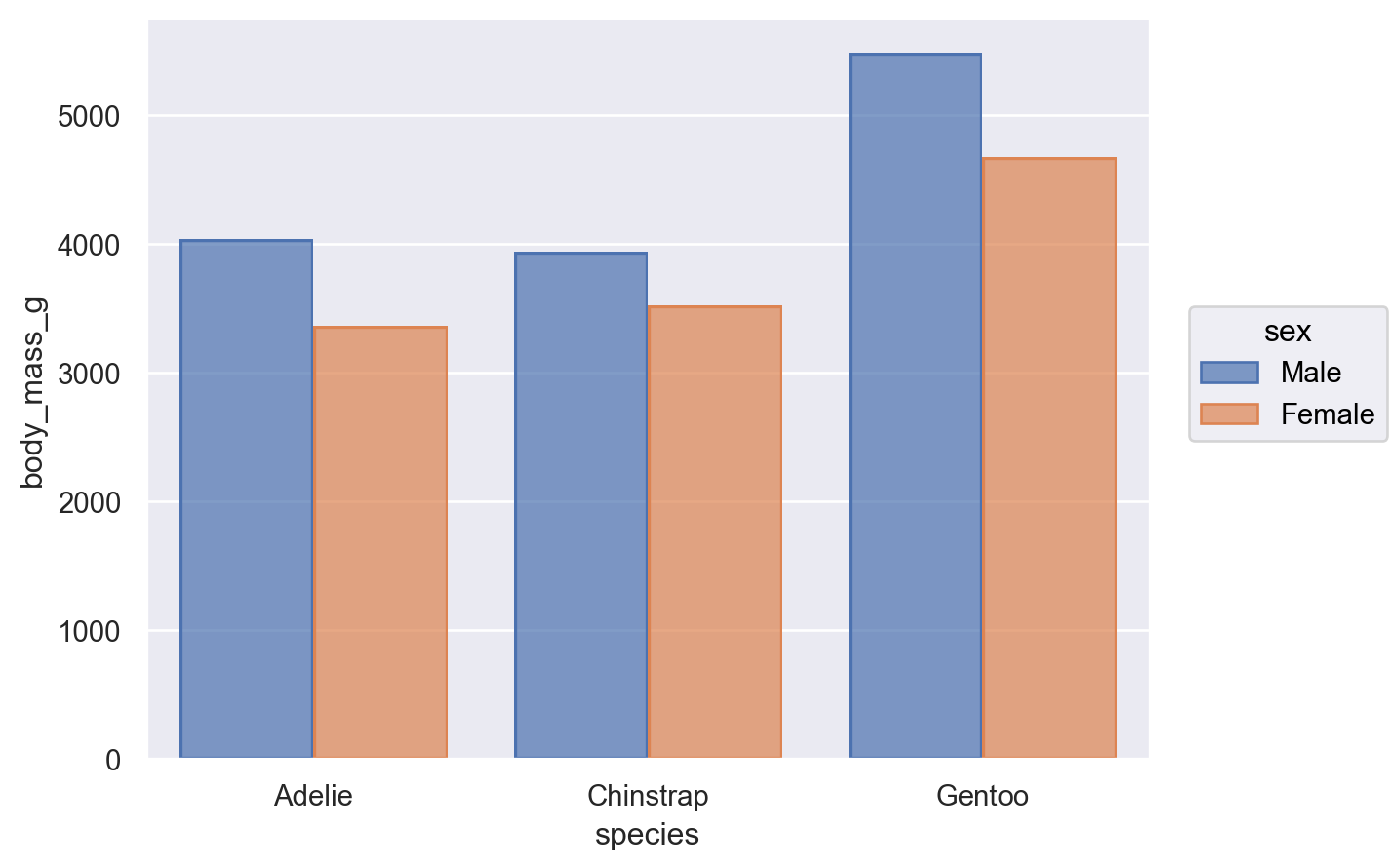The `Dodge` class is an example of a `Move` transformation, which is like a `Stat` but only adjusts `x` and `y` coordinates. The `Move` classes can be applied with any mark, and it’s not necessary to use a `Stat` first:

```(
so.Plot(penguins, x="species", y="body_mass_g", color="sex")
)
```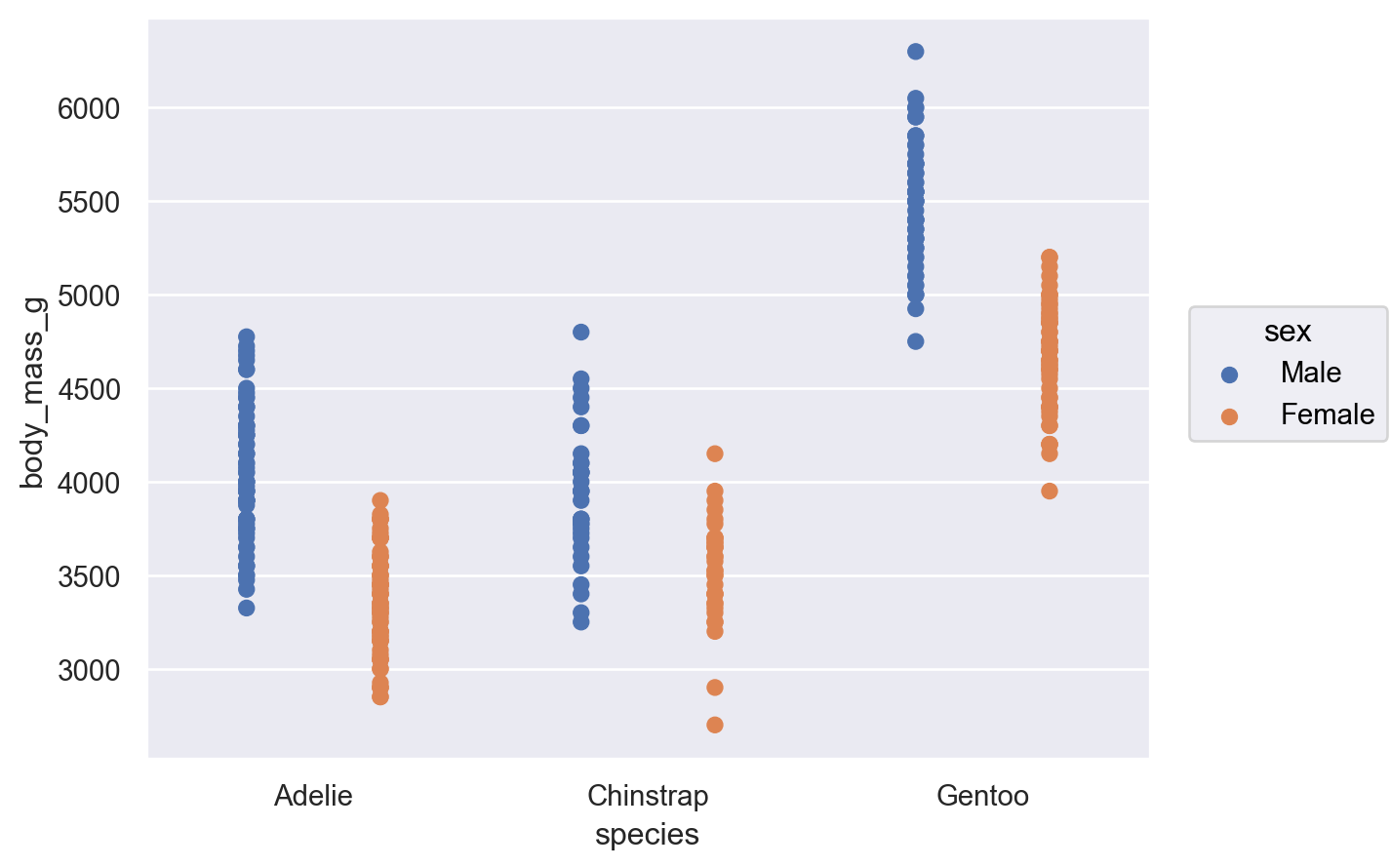It’s also possible to apply multiple `Move` operations in sequence:

```(
so.Plot(penguins, x="species", y="body_mass_g", color="sex")
)
```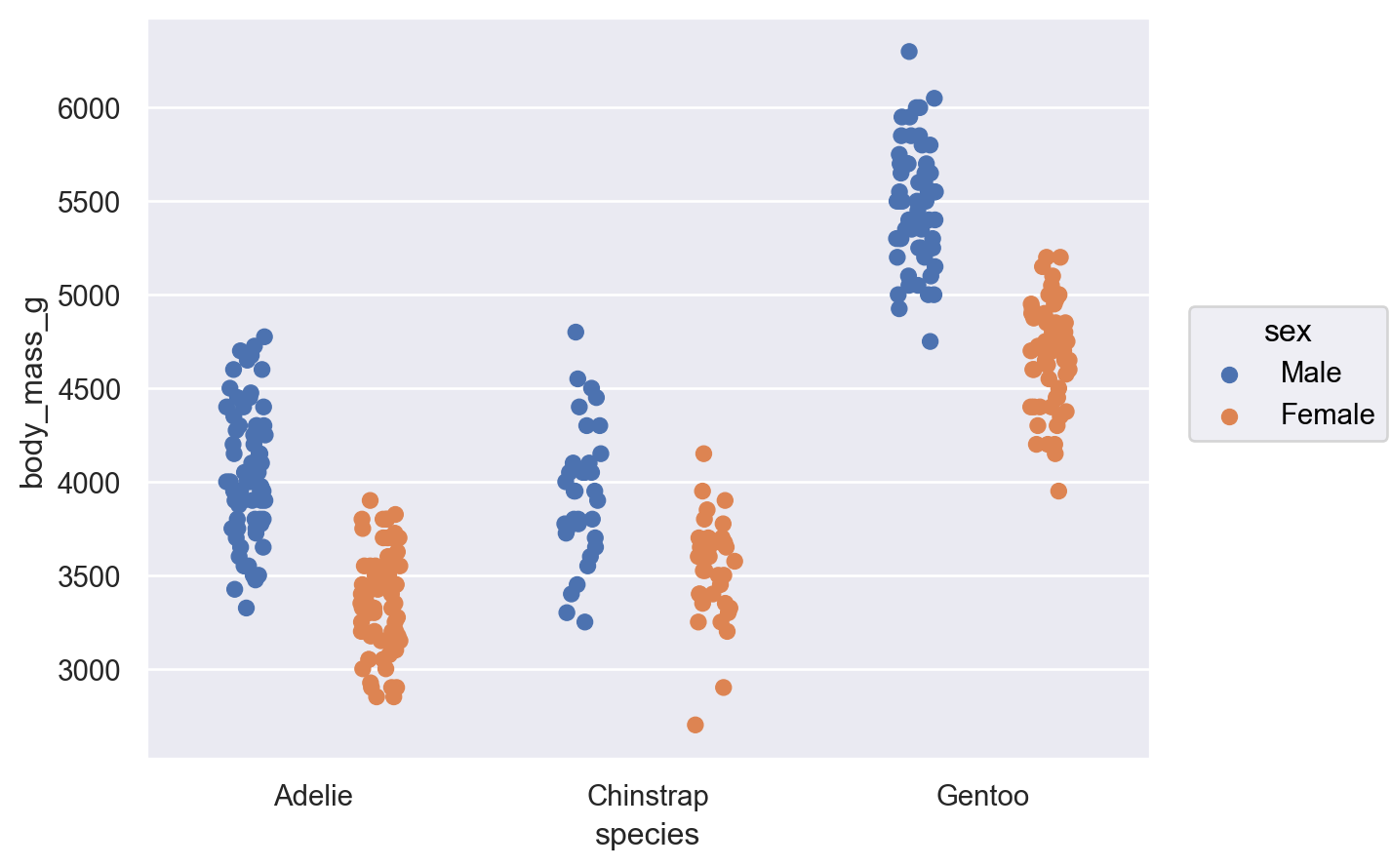### Creating variables through transformation#

The `Agg` stat requires both `x` and `y` to already be defined, but variables can also be created through statistical transformation. For example, the `Hist` stat requires only one of `x` or `y` to be defined, and it will create the other by counting observations:

```(
so.Plot(penguins, x="species")
)
```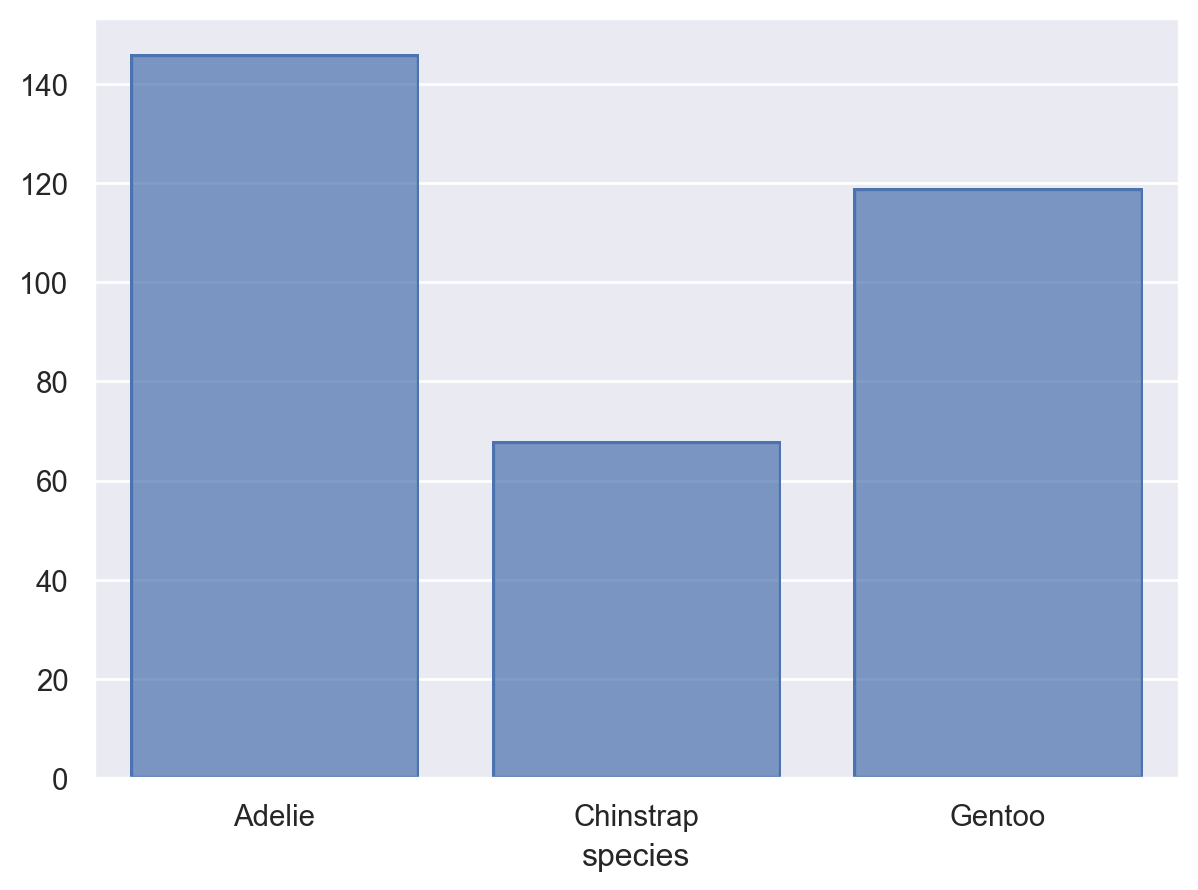The `Hist` stat will also create new `x` values (by binning) when given numeric data:

```(
so.Plot(penguins, x="flipper_length_mm")
)
```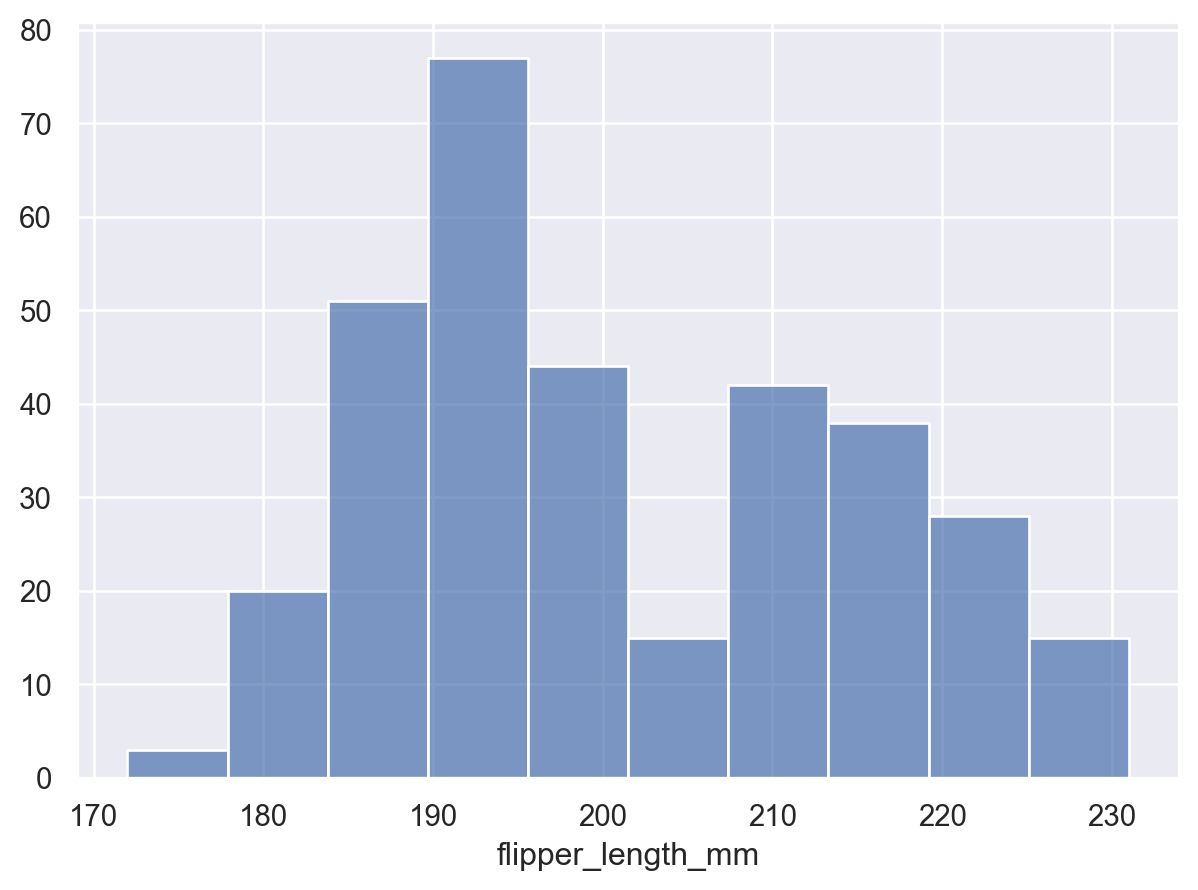Notice how we used `Bars`, rather than `Bar` for the plot with the continuous `x` axis. These two marks are related, but `Bars` has different defaults and works better for continuous histograms. It also produces a different, more efficient matplotlib artist. You will find the pattern of singular/plural marks elsewhere. The plural version is typically optimized for cases with larger numbers of marks.

Some transforms accept both `x` and `y`, but add interval data for each coordinate. This is particularly relevant for plotting error bars after aggregating:

```(
so.Plot(penguins, x="body_mass_g", y="species", color="sex")
)
```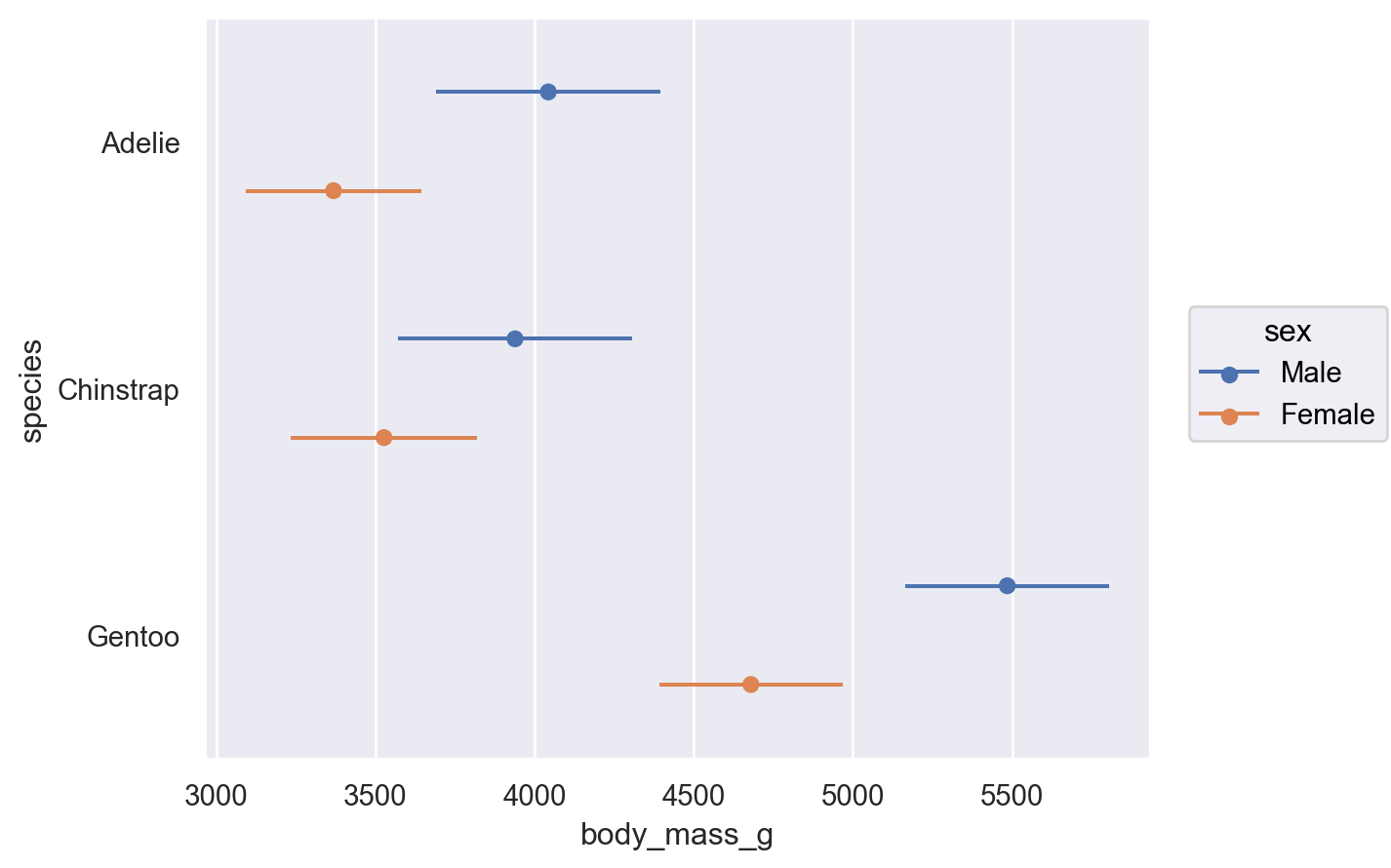### Orienting marks and transforms#

When aggregating, dodging, and drawing a bar, the `x` and `y` variables are treated differently. Each operation has the concept of an orientation. The `Plot` tries to determine the orientation automatically based on the data types of the variables. For instance, if we flip the assignment of `species` and `body_mass_g`, we’ll get the same plot, but oriented horizontally:

```(
so.Plot(penguins, x="body_mass_g", y="species", color="sex")
)
```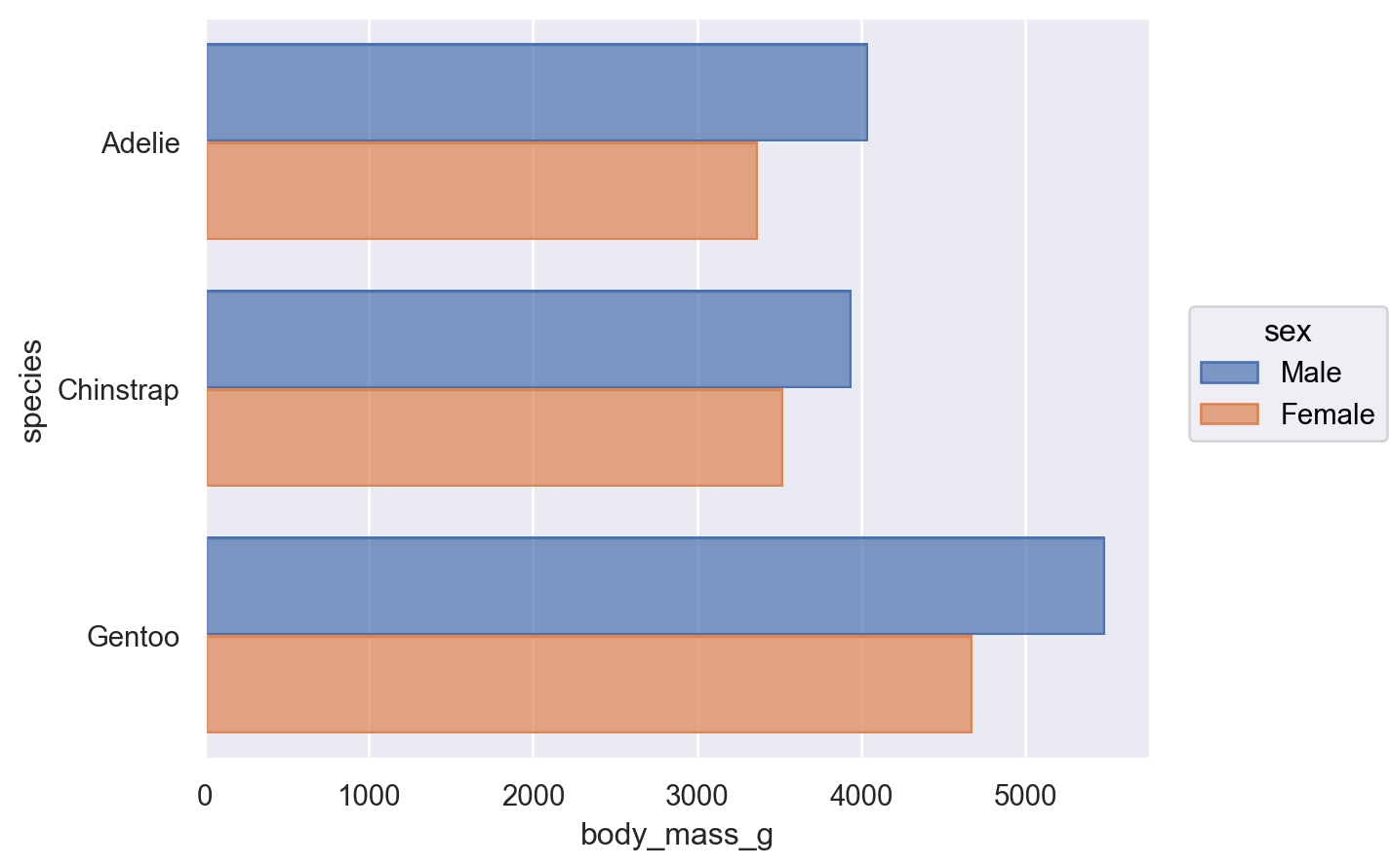Sometimes, the correct orientation is ambiguous, as when both the `x` and `y` variables are numeric. In these cases, you can be explicit by passing the `orient` parameter to `Plot.add()`:

```(
so.Plot(tips, x="total_bill", y="size", color="time")
)
```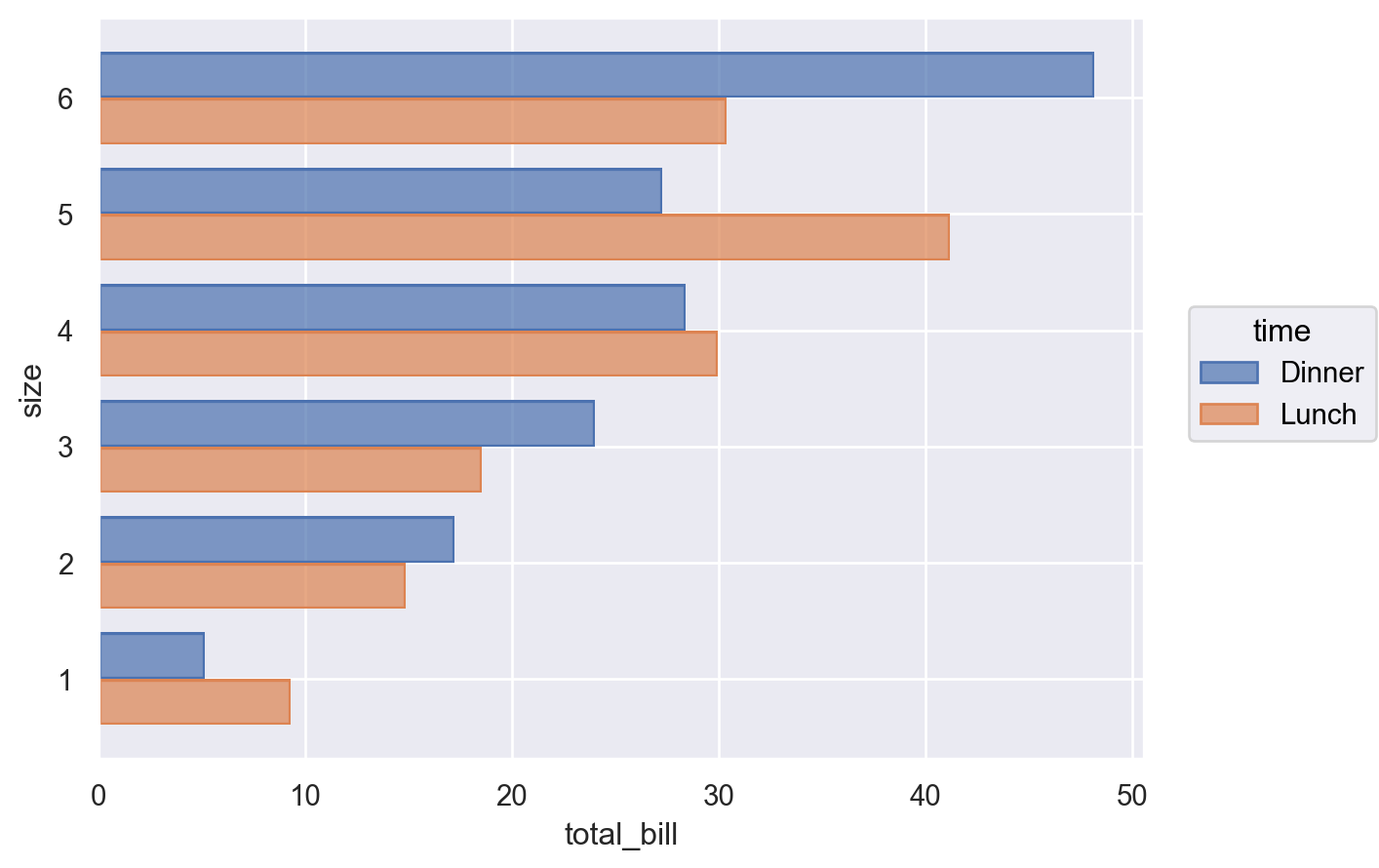## Building and displaying the plot#

Most examples this far have produced a single subplot with just one kind of mark on it. But `Plot` does not limit you to this.

More complex single-subplot graphics can be created by calling `Plot.add()` repeatedly. Each time it is called, it defines a layer in the plot. For example, we may want to add a scatterplot (now using `Dots`) and then a regression fit:

```(
so.Plot(tips, x="total_bill", y="tip")
)
```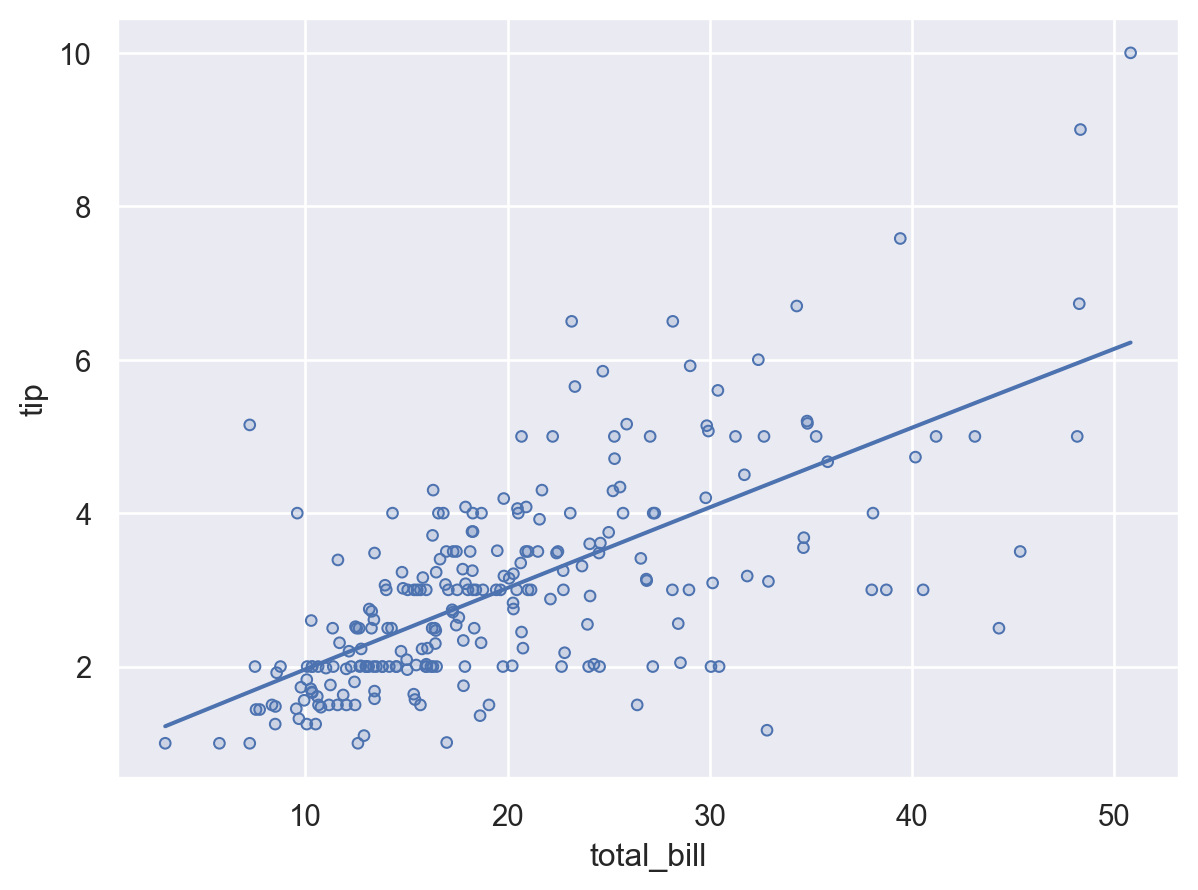Variable mappings that are defined in the `Plot` constructor will be used for all layers:

```(
so.Plot(tips, x="total_bill", y="tip", color="time")
)
```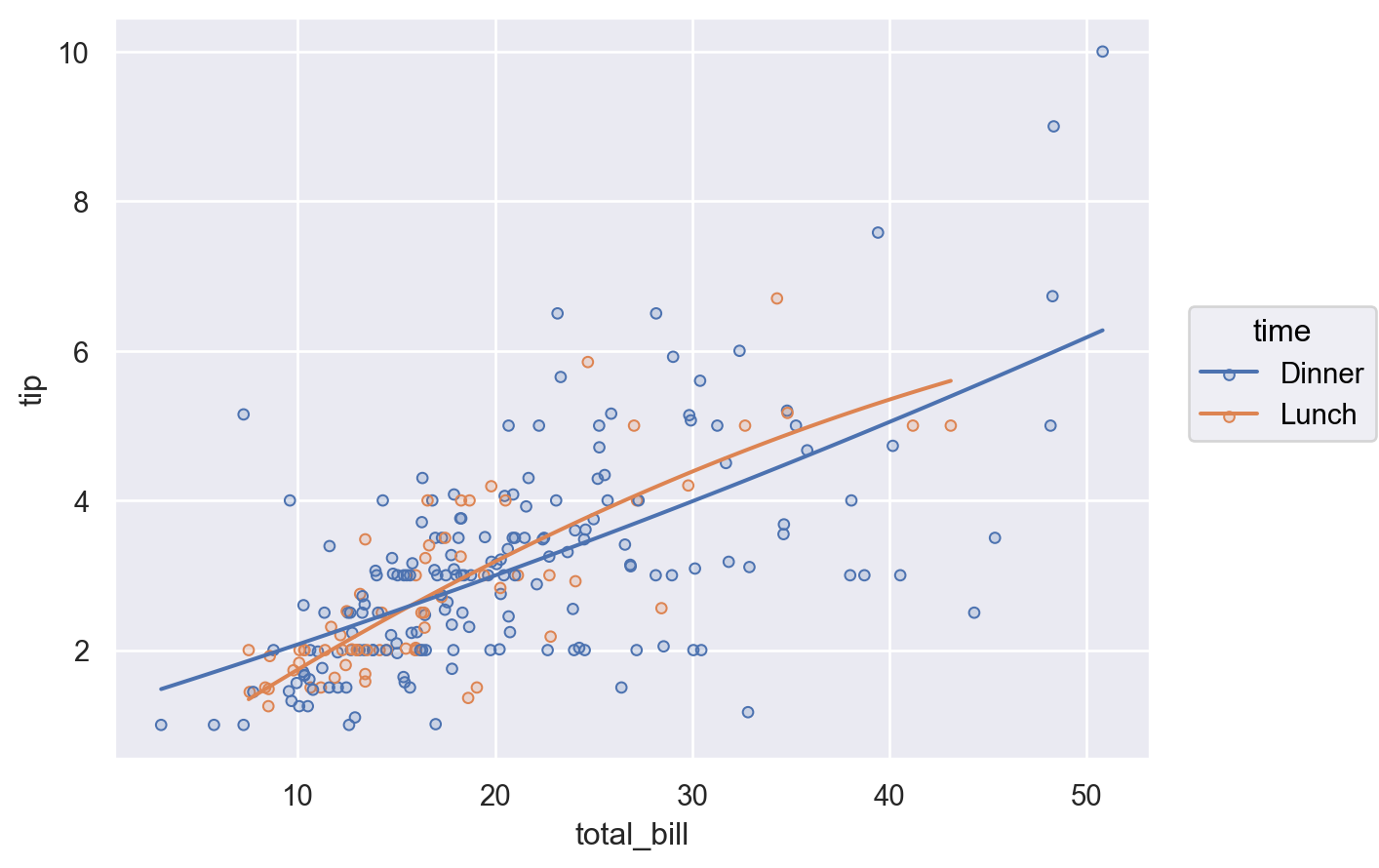### Layer-specific mappings#

You can also define a mapping such that it is used only in a specific layer. This is accomplished by defining the mapping within the call to `Plot.add` for the relevant layer:

```(
so.Plot(tips, x="total_bill", y="tip")
)
```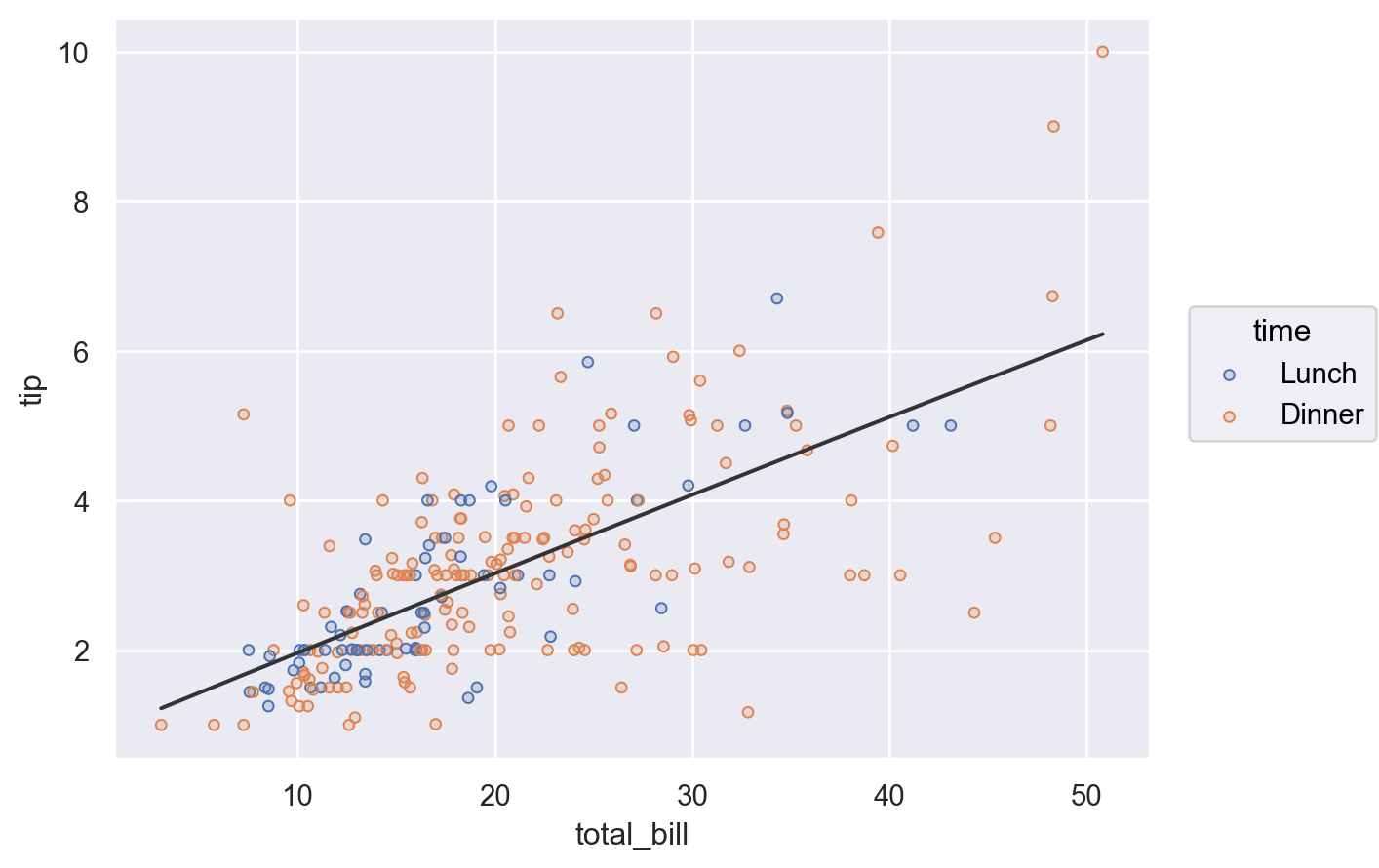Alternatively, define the layer for the entire plot, but remove it from a specific layer by setting the variable to `None`:

```(
so.Plot(tips, x="total_bill", y="tip", color="time")
)
```To recap, there are three ways to specify the value of a mark property: (1) by mapping a variable in all layers, (2) by mapping a variable in a specific layer, and (3) by setting the property directly:### Faceting and pairing subplots#

As with seaborn’s figure-level functions (`seaborn.displot()`, `seaborn.catplot()`, etc.), the `Plot` interface can also produce figures with multiple “facets”, or subplots containing subsets of data. This is accomplished with the `Plot.facet()` method:

```(
so.Plot(penguins, x="flipper_length_mm")
.facet("species")
)
```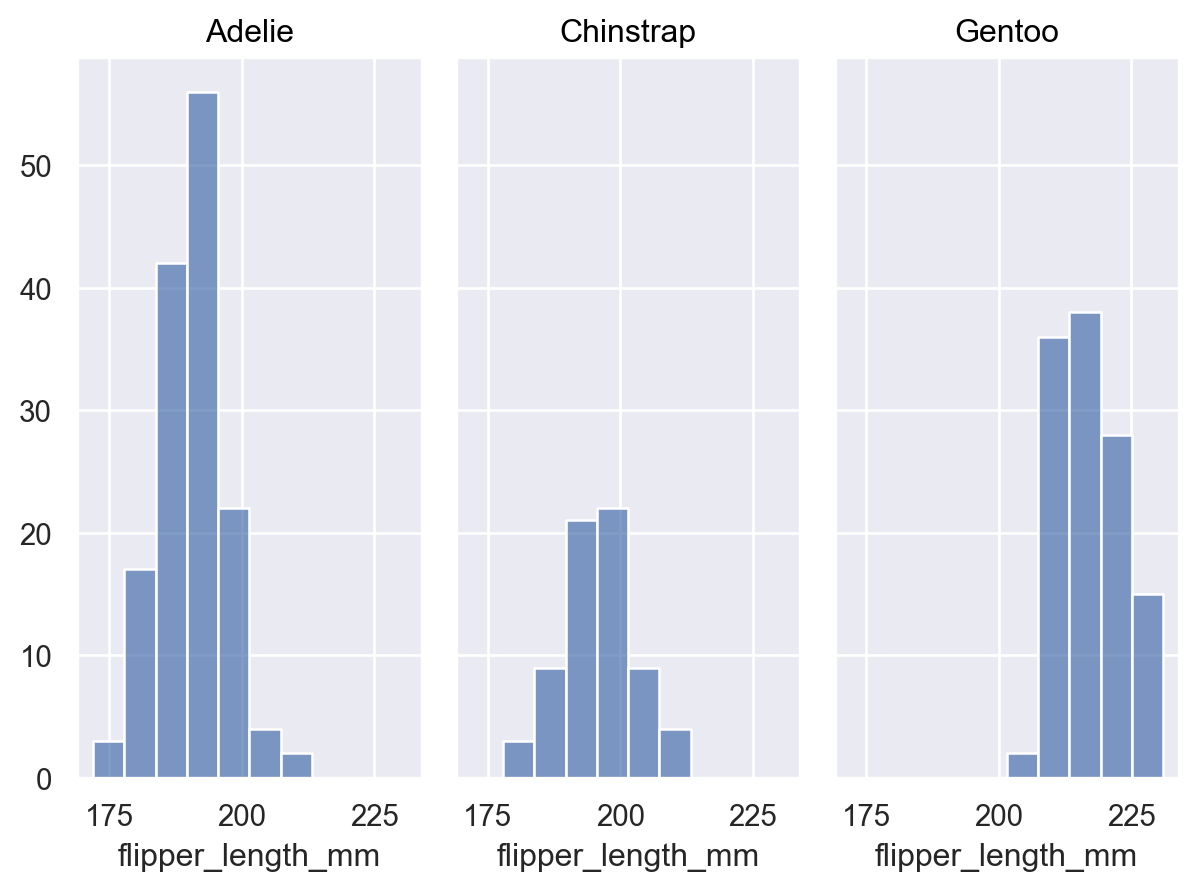Call `Plot.facet()` with the variables that should be used to define the columns and/or rows of the plot:

```(
so.Plot(penguins, x="flipper_length_mm")
.facet(col="species", row="sex")
)
```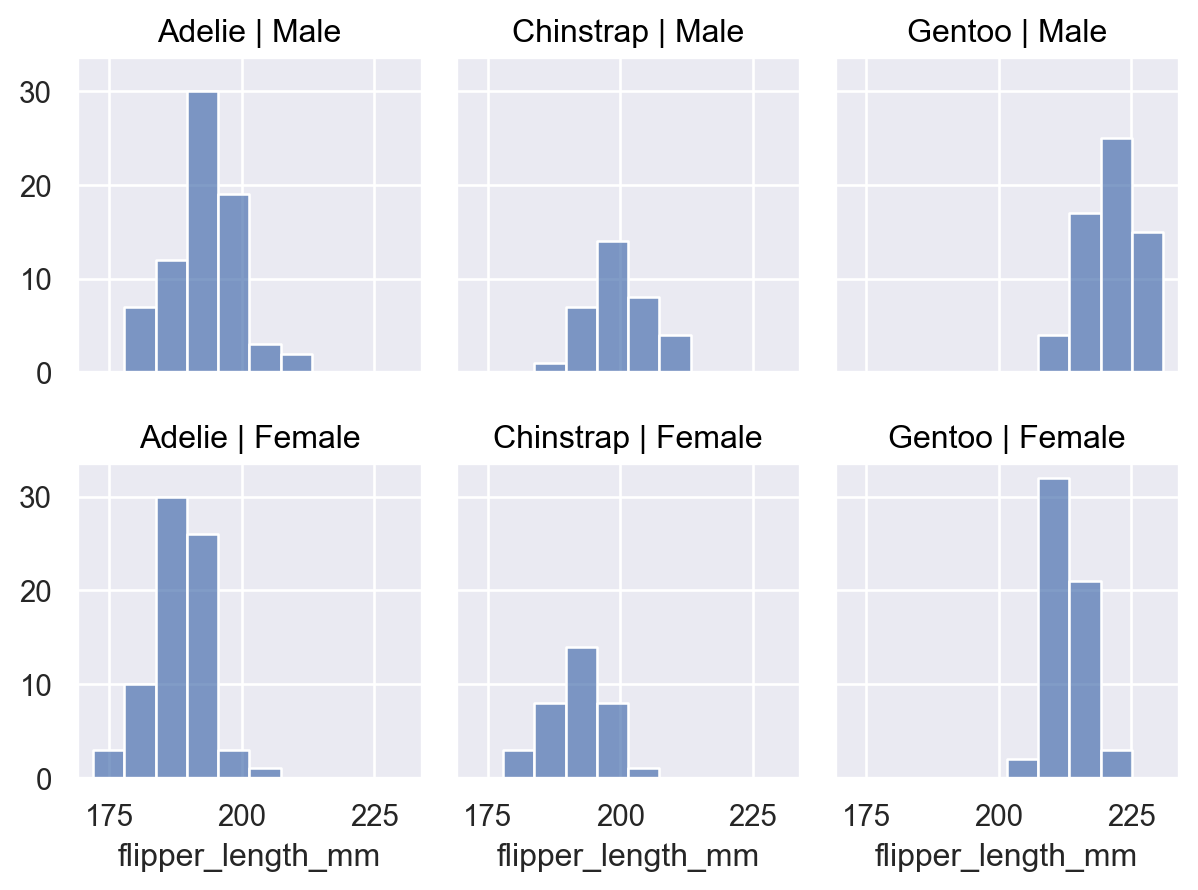You can facet using a variable with a larger number of levels by “wrapping” across the other dimension:

```(
so.Plot(healthexp, x="Year", y="Life_Expectancy")
.facet(col="Country", wrap=3)
)
```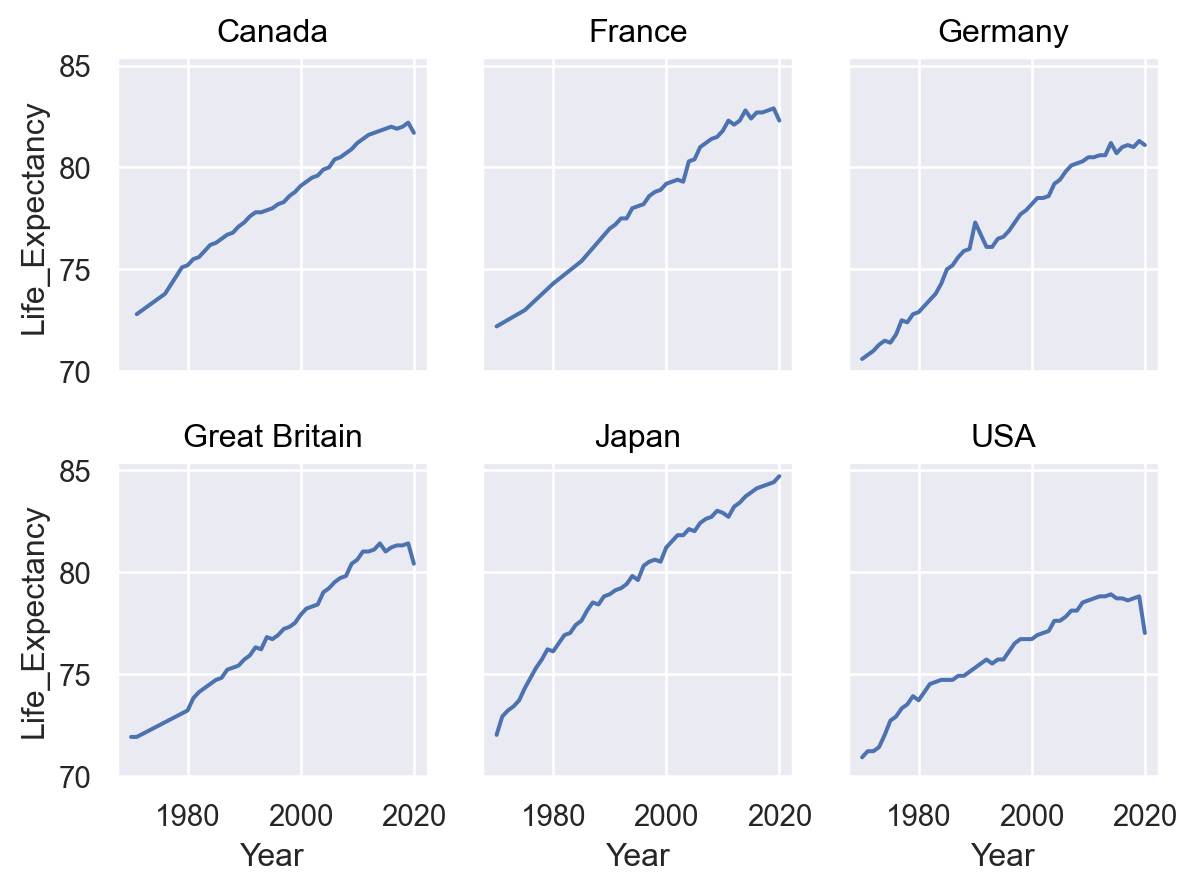All layers will be faceted unless you explicitly exclude them, which can be useful for providing additional context on each subplot:

```(
so.Plot(healthexp, x="Year", y="Life_Expectancy")
.facet("Country", wrap=3)
)
```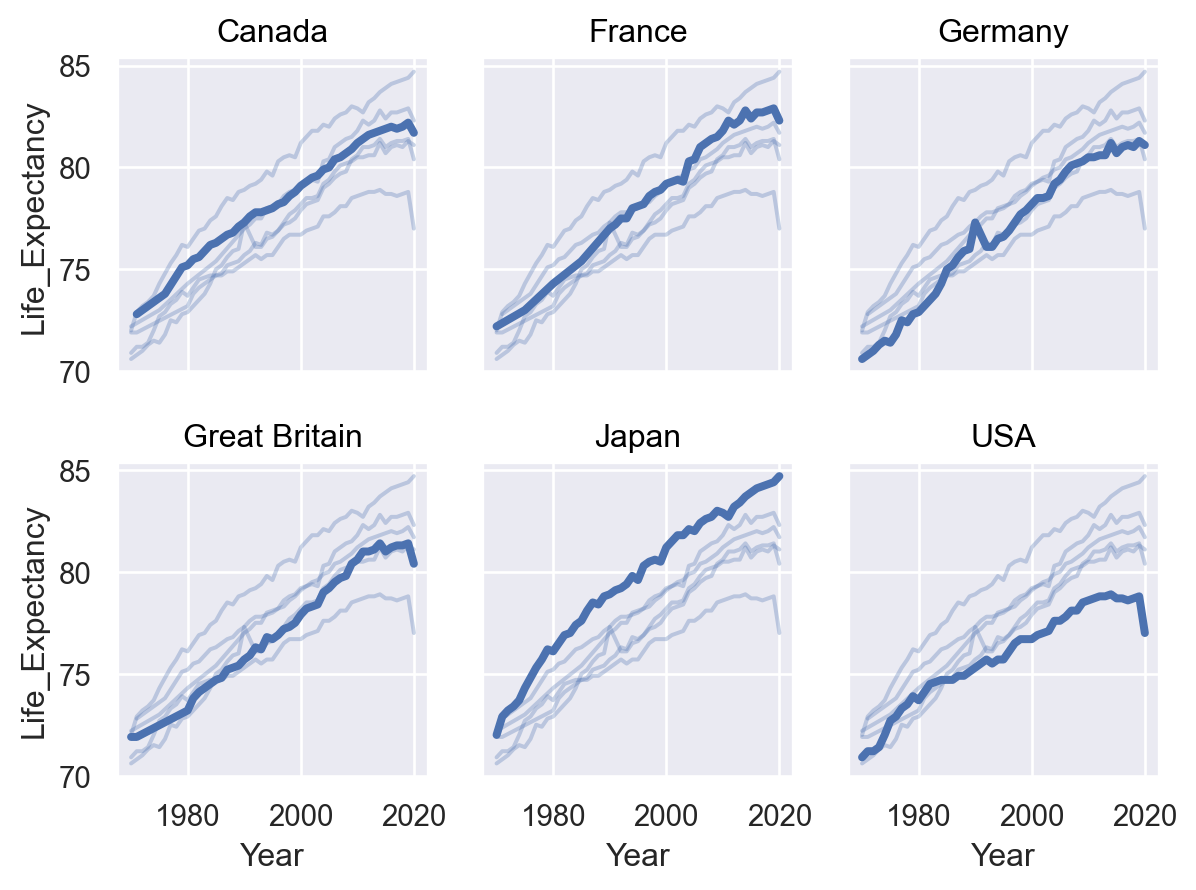An alternate way to produce subplots is `Plot.pair()`. Like `seaborn.PairGrid`, this draws all of the data on each subplot, using different variables for the x and/or y coordinates:

```(
so.Plot(penguins, y="body_mass_g", color="species")
.pair(x=["bill_length_mm", "bill_depth_mm"])
)
```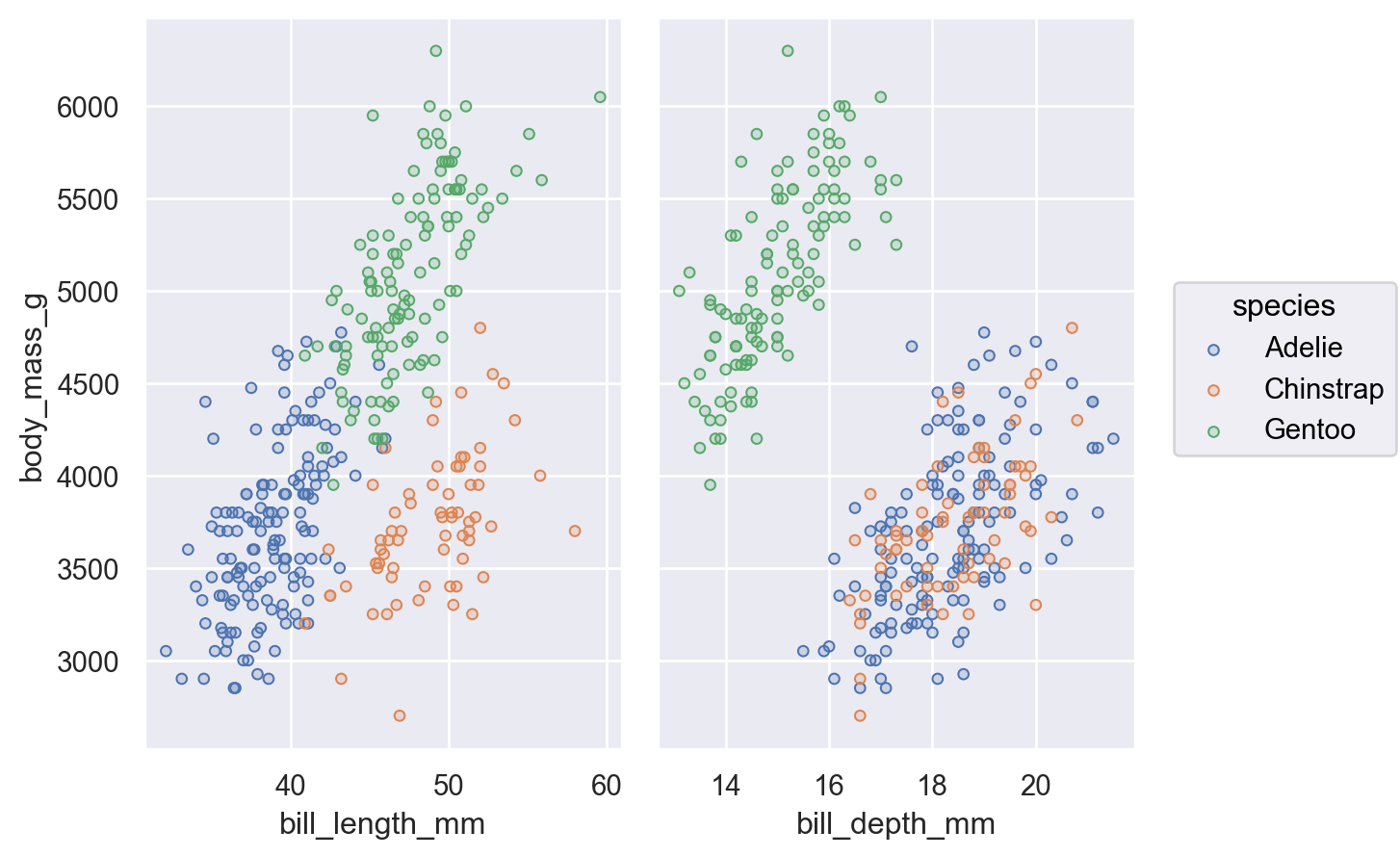You can combine faceting and pairing so long as the operations add subplots on opposite dimensions:

```(
so.Plot(penguins, y="body_mass_g", color="species")
.pair(x=["bill_length_mm", "bill_depth_mm"])
.facet(row="sex")
)
```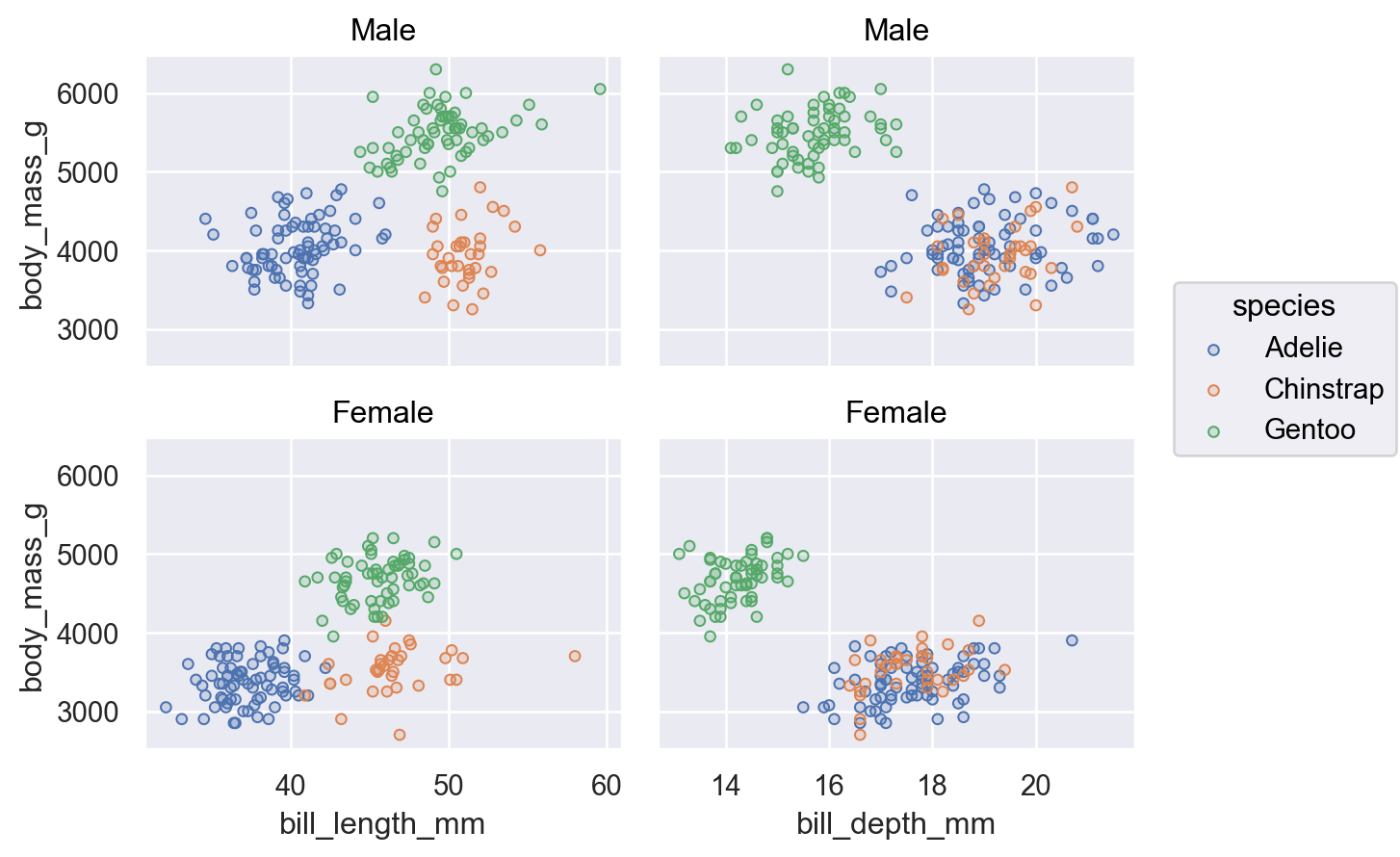### Integrating with matplotlib#

There may be cases where you want multiple subplots to appear in a figure with a more complex structure than what `Plot.facet()` or `Plot.pair()` can provide. The current solution is to delegate figure setup to matplotlib and to supply the matplotlib object that `Plot` should use with the `Plot.on()` method. This object can be either a `matplotlib.axes.Axes`, `matplotlib.figure.Figure`, or `matplotlib.figure.SubFigure`; the latter is most useful for constructing bespoke subplot layouts:

```f = mpl.figure.Figure(figsize=(8, 4))
sf1, sf2 = f.subfigures(1, 2)
(
so.Plot(penguins, x="body_mass_g", y="flipper_length_mm")
.on(sf1)
.plot()
)
(
so.Plot(penguins, x="body_mass_g")
.facet(row="sex")
.on(sf2)
.plot()
)
```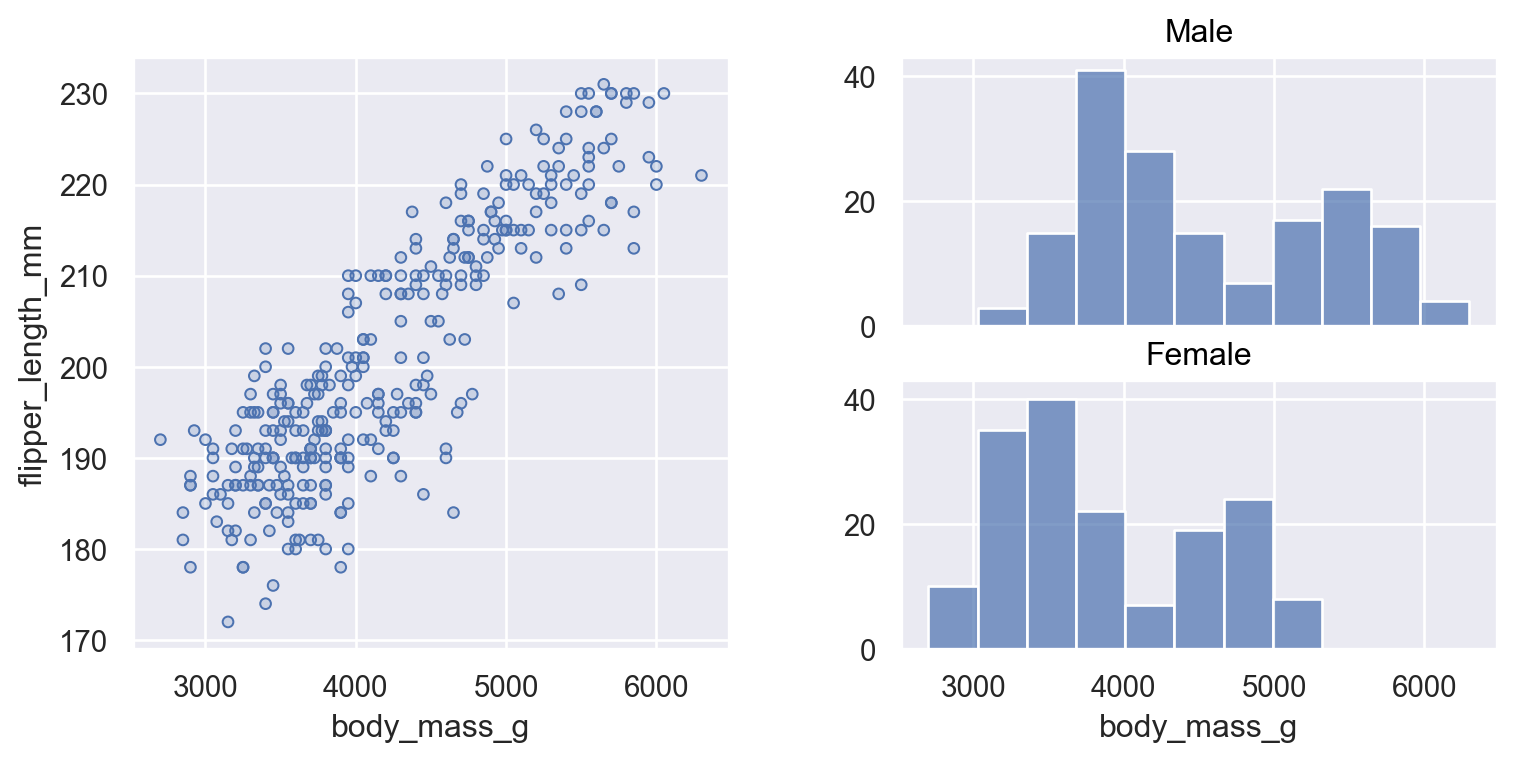### Building and displaying the plot#

An important thing to know is that `Plot` methods clone the object they are called on and return that clone instead of updating the object in place. This means that you can define a common plot spec and then produce several variations on it.

So, take this basic specification:

```p = so.Plot(healthexp, "Year", "Spending_USD", color="Country")
```

We could use it to draw a line plot:

```p.add(so.Line())
```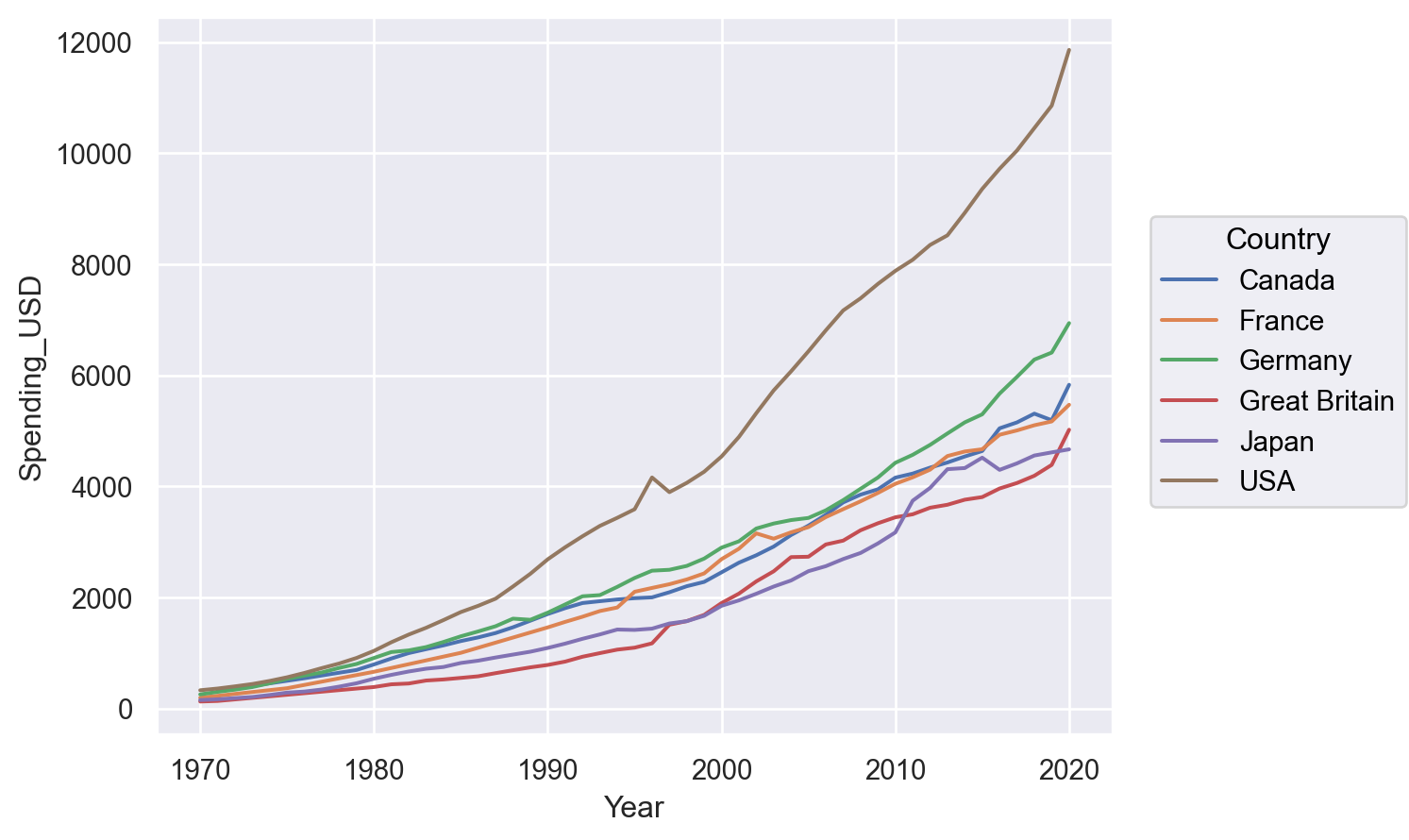Or perhaps a stacked area plot:

```p.add(so.Area(), so.Stack())
```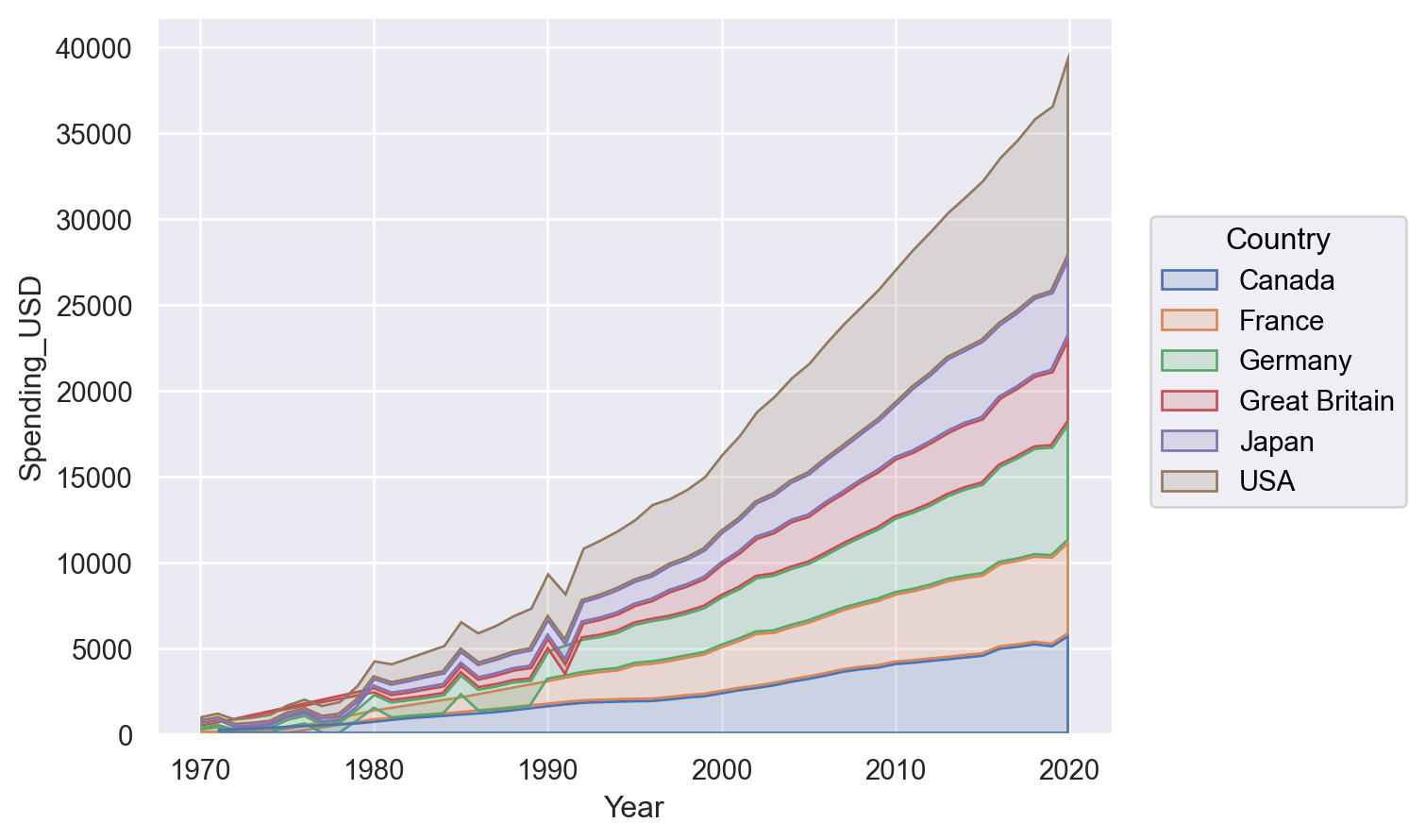The `Plot` methods are fully declarative. Calling them updates the plot spec, but it doesn’t actually do any plotting. One consequence of this is that methods can be called in any order, and many of them can be called multiple times.

When does the plot actually get rendered? `Plot` is optimized for use in notebook environments. The rendering is automatically triggered when the `Plot` gets displayed in the Jupyter REPL. That’s why we didn’t see anything in the example above, where we defined a `Plot` but assigned it to `p` rather than letting it return out to the REPL.

To see a plot in a notebook, either return it from the final line of a cell or call Jupyter’s built-in `display` function on the object. The notebook integration bypasses `matplotlib.pyplot` entirely, but you can use its figure-display machinery in other contexts by calling `Plot.show()`.

You can also save the plot to a file (or buffer) by calling `Plot.save()`.

## Customizing the appearance#

The new interface aims to support a deep amount of customization through `Plot`, reducing the need to switch gears and use matplotlib functionality directly. (But please be patient; not all of the features needed to achieve this goal have been implemented!)

### Parameterizing scales#

All of the data-dependent properties are controlled by the concept of a `Scale` and the `Plot.scale()` method. This method accepts several different types of arguments. One possibility, which is closest to the use of scales in matplotlib, is to pass the name of a function that transforms the coordinates:

```(
so.Plot(diamonds, x="carat", y="price")
.scale(y="log")
)
```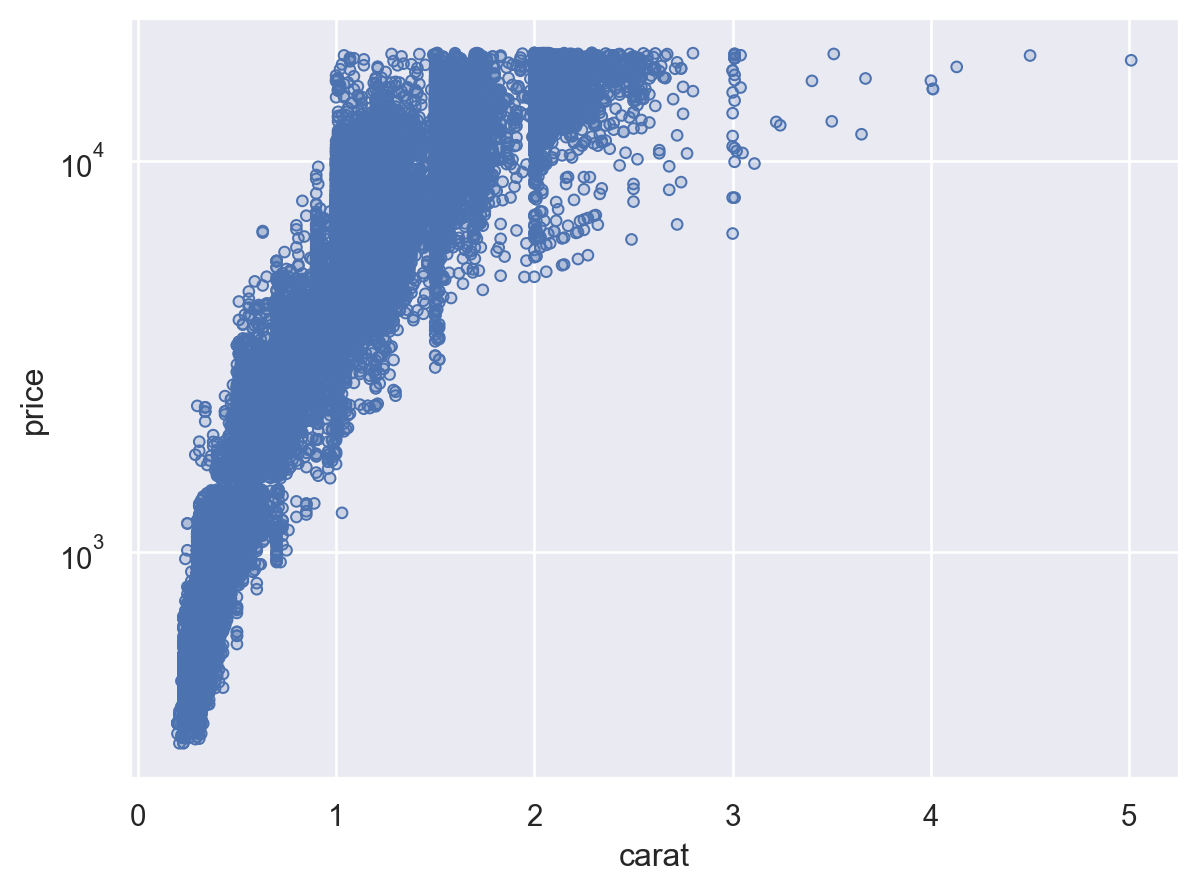`Plot.scale()` can also control the mappings for semantic properties like `color`. You can directly pass it any argument that you would pass to the `palette` parameter in seaborn’s function interface:

```(
so.Plot(diamonds, x="carat", y="price", color="clarity")
.scale(color="flare")
)
```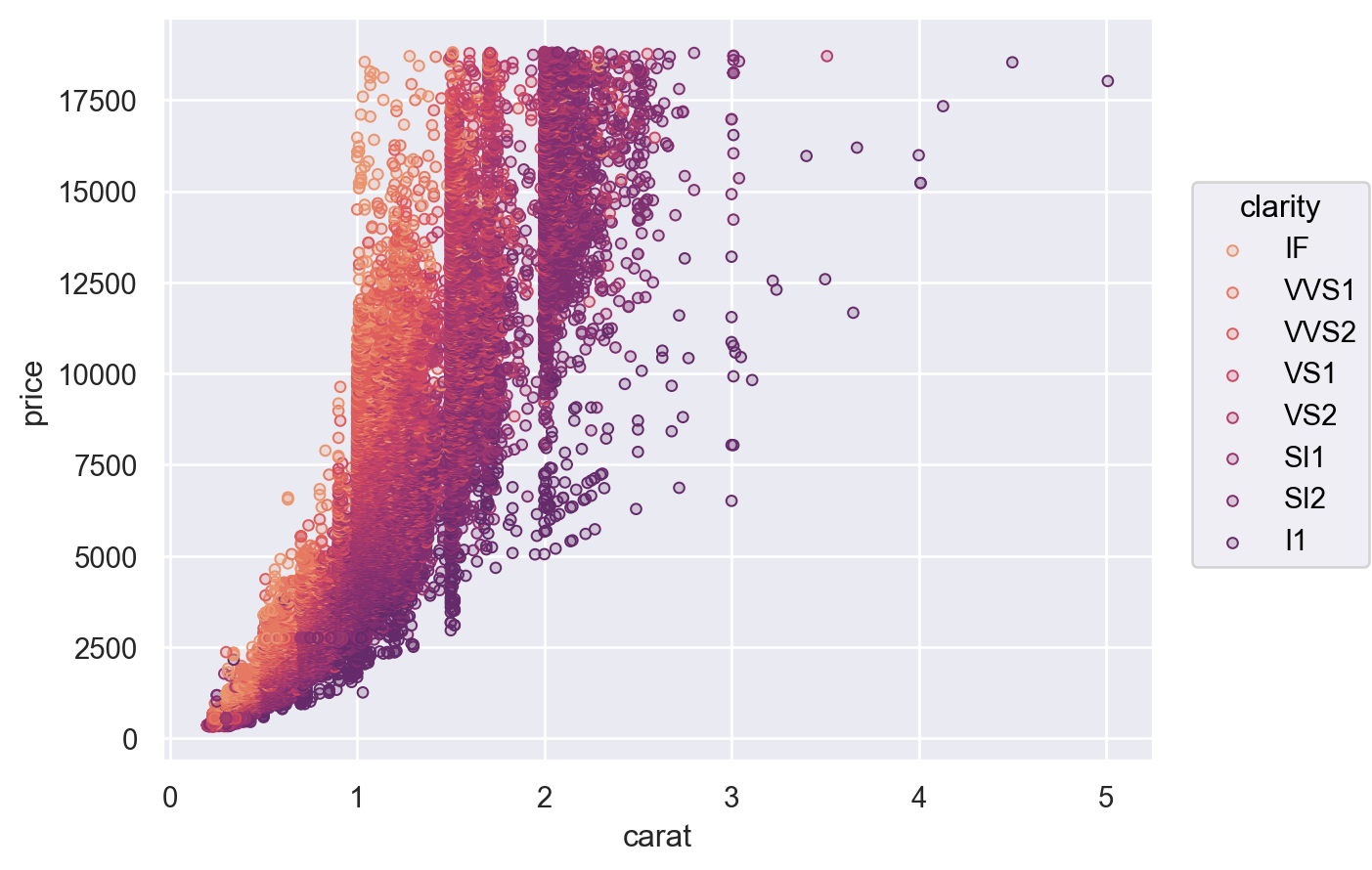Another option is to provide a tuple of `(min, max)` values, controlling the range that the scale should map into. This works both for numeric properties and for colors:

```(
so.Plot(diamonds, x="carat", y="price", color="clarity", pointsize="carat")
.scale(color=("#88c", "#555"), pointsize=(2, 10))
)
```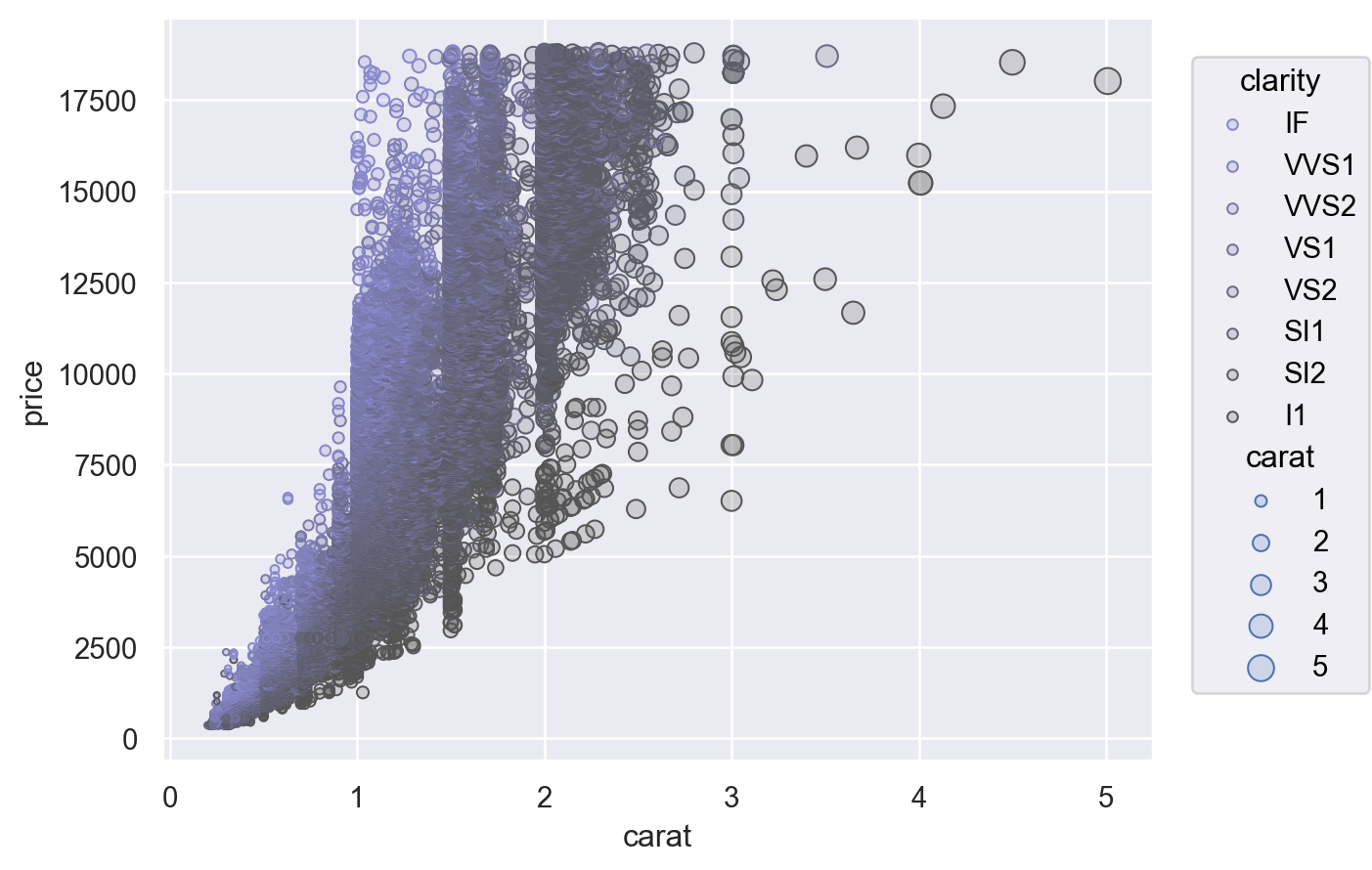For additional control, you can pass a `Scale` object. There are several different types of `Scale`, each with appropriate parameters. For example, `Continuous` lets you define the input domain (`norm`), the output range (`values`), and the function that maps between them (`trans`), while `Nominal` allows you to specify an ordering:

```(
so.Plot(diamonds, x="carat", y="price", color="carat", marker="cut")
.scale(
color=so.Continuous("crest", norm=(0, 3), trans="sqrt"),
marker=so.Nominal(["o", "+", "x"], order=["Ideal", "Premium", "Good"]),
)
)
```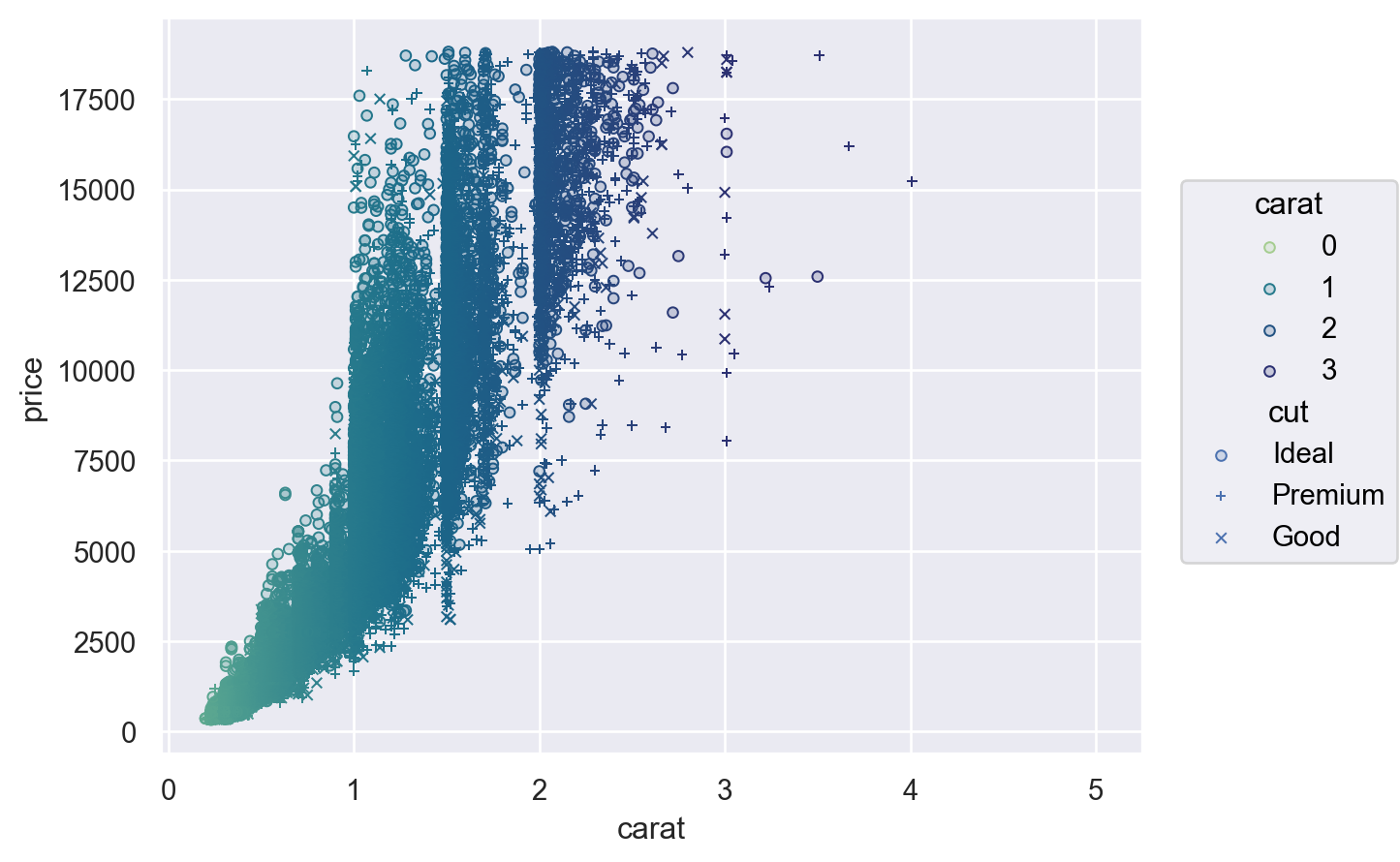### Customizing legends and ticks#

The `Scale` objects are also how you specify which values should appear as tick labels / in the legend, along with how they appear. For example, the `Continuous.tick()` method lets you control the density or locations of the ticks, and the `Continuous.label()` method lets you modify the format:

```(
so.Plot(diamonds, x="carat", y="price", color="carat")
.scale(
x=so.Continuous().tick(every=0.5),
y=so.Continuous().label(like="\${x:.0f}"),
color=so.Continuous().tick(at=[1, 2, 3, 4]),
)
)
```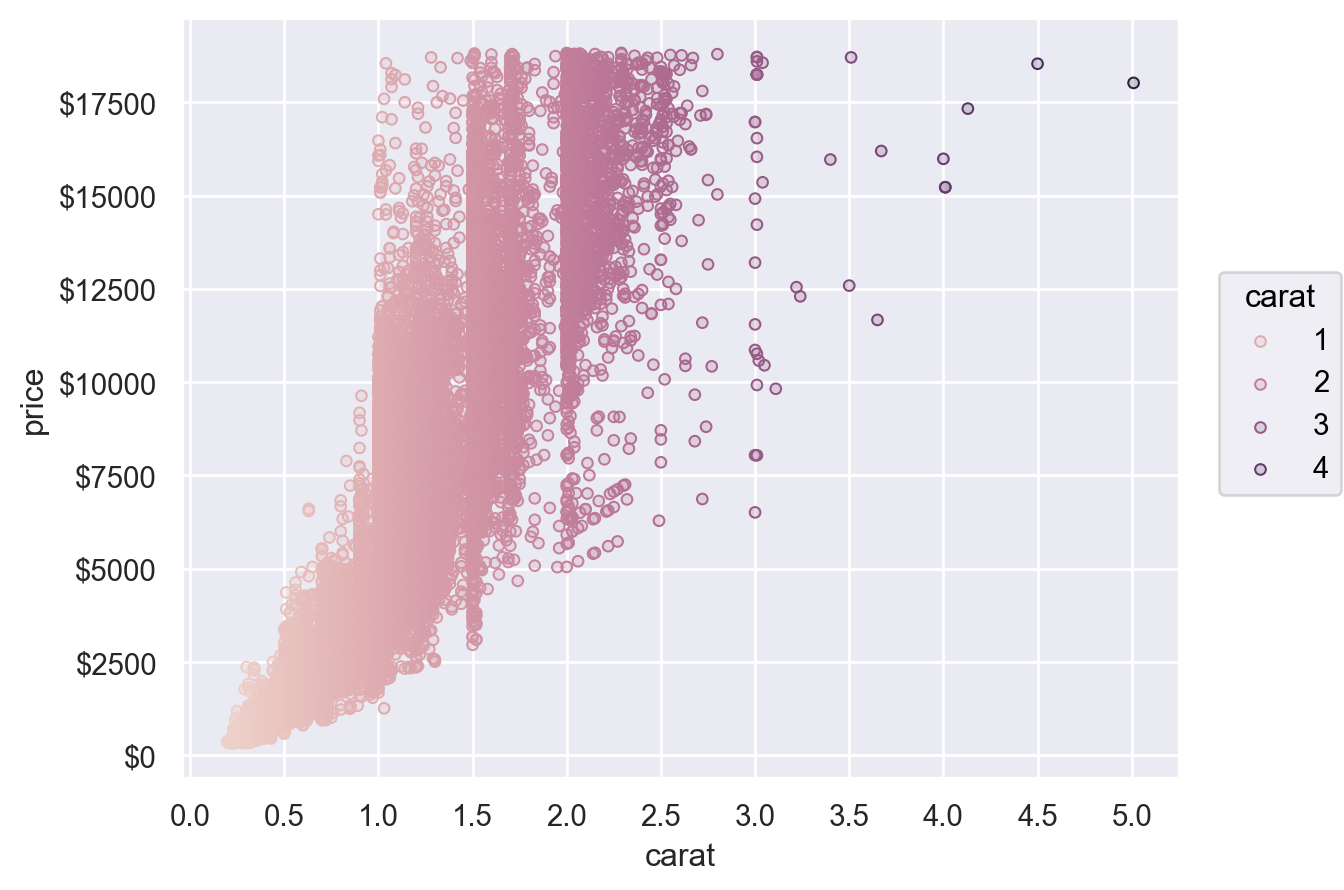### Customizing limits, labels, and titles#

`Plot` has a number of methods for simple customization, including `Plot.label()`, `Plot.limit()`, and `Plot.share()`:

```(
so.Plot(penguins, x="body_mass_g", y="species", color="island")
.facet(col="sex")
.share(x=False)
.limit(y=(2.5, -.5))
.label(
x="Body mass (g)", y="",
color=str.capitalize,
title="{} penguins".format,
)
)
```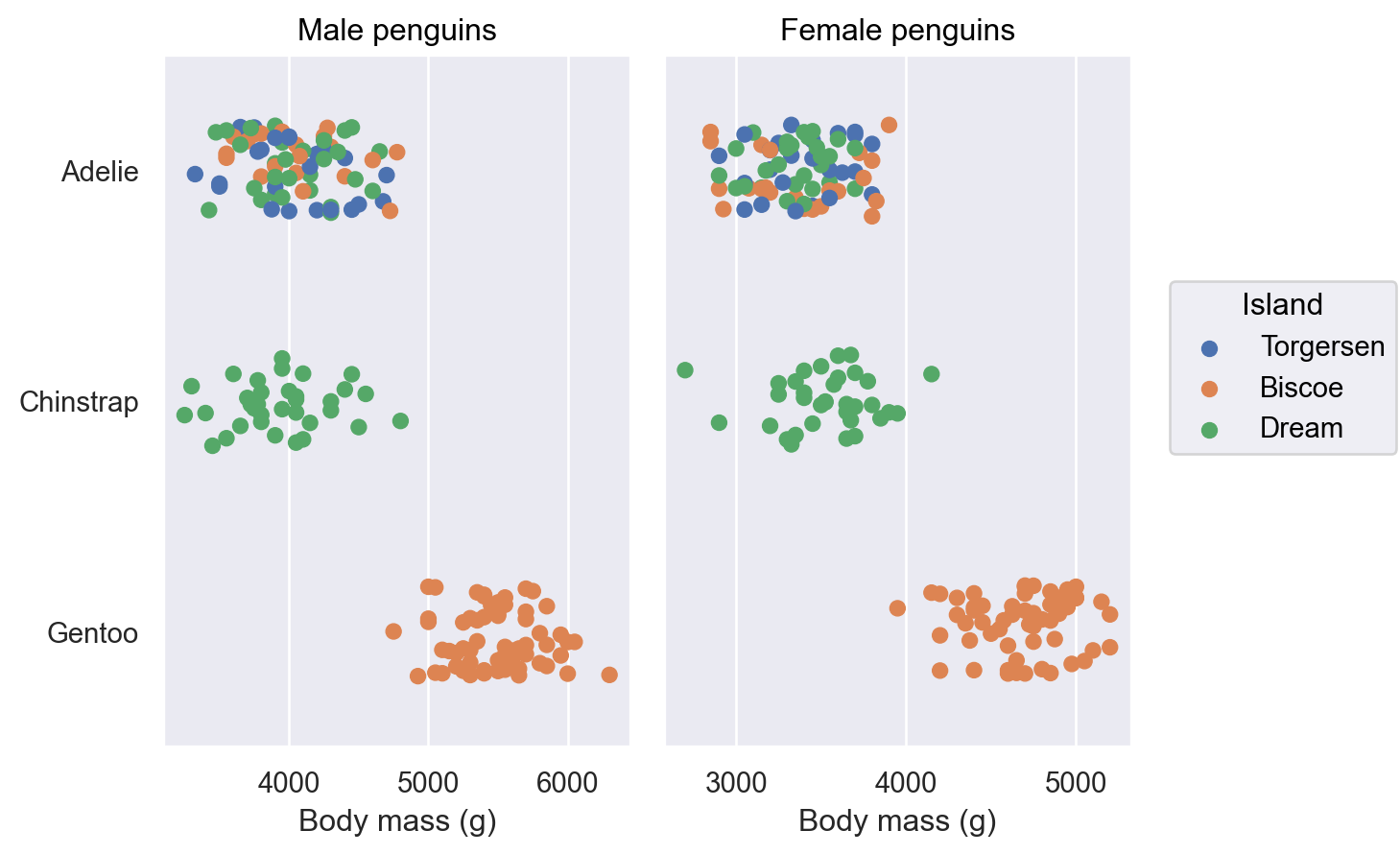### Theme customization#

Finally, `Plot` supports data-independent theming through the `Plot.theme` method. Currently, this method accepts a dictionary of matplotlib rc parameters. You can set them directly and/or pass a package of parameters from seaborn’s theming functions:

```from seaborn import axes_style
theme_dict = {**axes_style("whitegrid"), "grid.linestyle": ":"}
so.Plot().theme(theme_dict)
```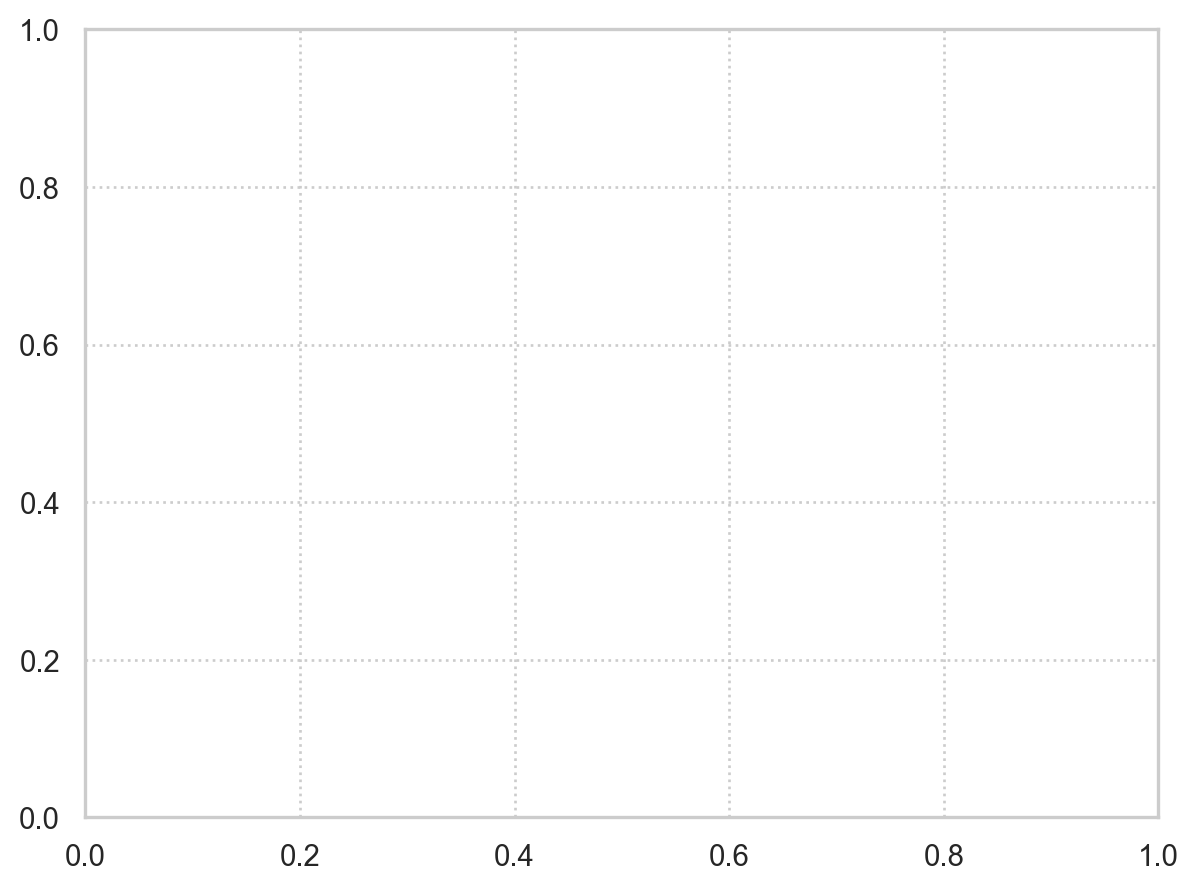To change the theme for all `Plot` instances, update the settings in `Plot.config`:

```so.Plot.config.theme.update(theme_dict)
```